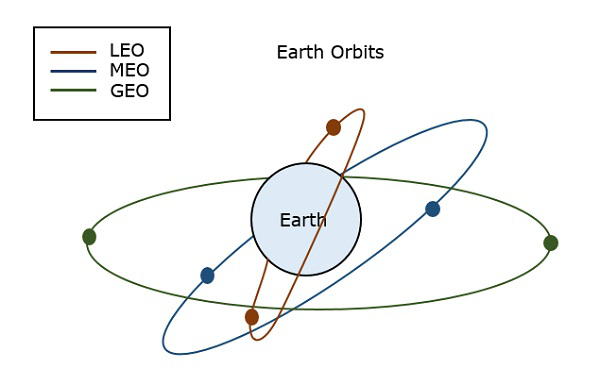# Principles of Communication - Introduction

The word communication arises from the Latin word “commūnicāre”, which means “to share”. Communication is the basic step for the exchange of information.

For example, a baby in a cradle, communicates with a cry that she needs her mother. A cow moos loudly when it is in danger. A person communicates with the help of a language. Communication is the bridge to share.

Communication can be defined as the process of exchange of information through means such as words, actions, signs, etc., between two or more individuals.

## Need for Communication

For any living being, while co-existing, there occurs the necessity of exchange of some information. Whenever a need for exchange of information arises, some means of communication should exist. While the means of communication, can be anything such as gestures, signs, symbols, or a language, the need for communication is inevitable.

Language and gestures play an important role in human communication, while sounds and actions are important for animal communication. However, when some message has to be conveyed, a communication has to be established.

## Parts of Communication System

Any system which provides communication, consists of the three important and basic parts as shown in the following figure.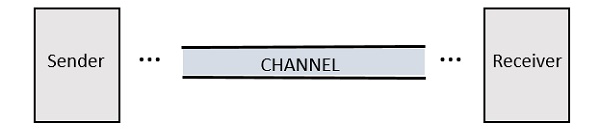• The Sender is the person who sends a message. It could be a transmitting station from where the signal is transmitted.

• The Channel is the medium through which the message signals travel to reach the destination.

• The Receiver is the person who receives the message. It could be a receiving station where the signal transmitted is received.

## What is a Signal?

Conveying an information by some means such as gestures, sounds, actions, etc., can be termed as signaling. Hence, a signal can be a source of energy which transmits some information. This signal helps to establish communication between a sender and a receiver.

An electrical impulse or an electromagnetic wave which travels a distance to convey a message, can be termed as a signal in communication systems.

Depending on their characteristics, signals are mainly classified into two types: Analog and Digital. Analog and Digital signals are further classified, as shown in the following figure.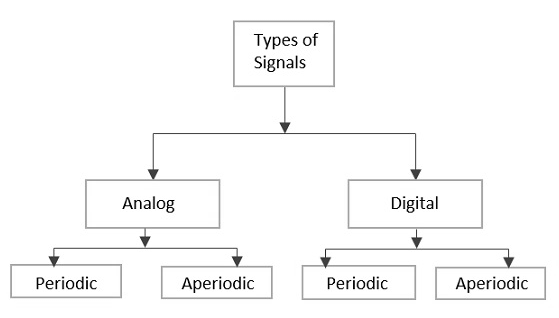## Analog Signal

A continuous time varying signal, which represents a time varying quantity can be termed as an Analog Signal. This signal keeps on varying with respect to time, according to the instantaneous values of the quantity, which represents it.

### Example

Let us consider, a tap that fills a tank of 100 liters capacity in an hour (6 am to 7 am). The portion of filling the tank is varied by the varying time. Which means, after 15 mins (6:15 am) the quarter portion of the tank gets filled, whereas at 6:45 am, 3/4th of the tank is filled.

If you try to plot the varying portions of water in the tank, according to the varying time, it would look like the following figure.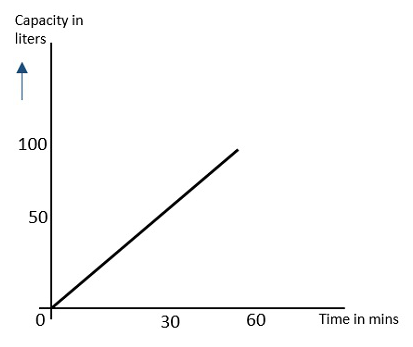As the resultant shown in this image varies (increases) according to time, this time varying quantity can be understood as Analog quantity. The signal which represents this condition with an inclined line in the figure, is an Analog Signal. The communication based on analog signals and analog values is called as Analog Communication.

## Digital Signal

A signal which is discrete in nature or which is non-continuous in form can be termed as a Digital signal. This signal has individual values, denoted separately, which are not based on the previous values, as if they are derived at that particular instant of time.

### Example

Let us consider a classroom having 20 students. If their attendance in a week is plotted, it would look like the following figure.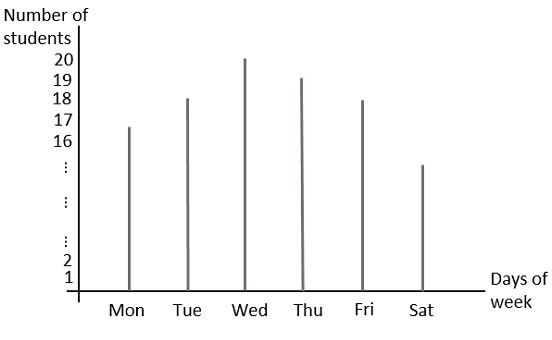In this figure, the values are separately stated. For instance, the attendance of the class on Wednesday is 20 whereas on Saturday is 15. These values can be considered individually and separately or discretely, hence they are called as discrete values.

The binary digits which has only 1s and 0s are mostly termed as digital values. Hence, the signals which represent 1s and 0s are also called as digital signals. The communication based on digital signals and digital values is called as Digital Communication.

## Periodic Signal

Any analog or digital signal, that repeats its pattern over a period of time, is called as a Periodic Signal. This signal has its pattern continued repeatedly and is easy to be assumed or to be calculated.

### Example

If we consider a machinery in an industry, the process that takes place one after the other is a continuous and repeat procedure. For example, procuring and grading the raw material, processing the material in batches, packing a load of products one after the other etc., follow a certain procedure repeatedly.

Such a process whether considered analog or digital, can be graphically represented as follows.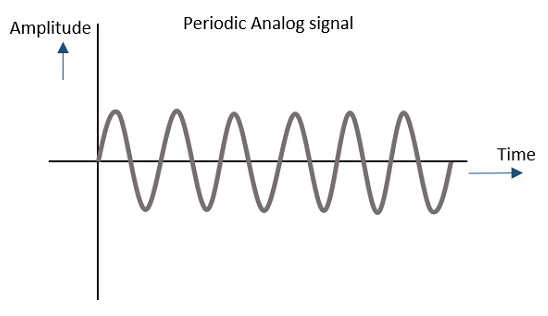## Aperiodic Signal

Any analog or digital signal, that doesn’t repeat its pattern over a period of time, is called as Aperiodic Signal. This signal has its pattern continued but the pattern is not repeated and is not so easy to be assumed or to be calculated.

### Example

The daily routine of a person, if considered, consists of many types of works which take different time intervals for different works. The time interval or the work doesn’t continuously repeat. For example, a person will not continuously brush his teeth from morning to night, that too with the same time period.

Such a process whether considered analog or digital, can be graphically represented as follows.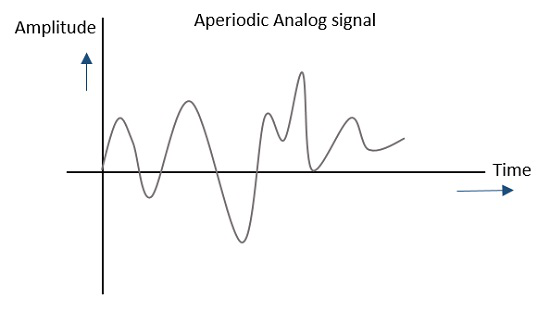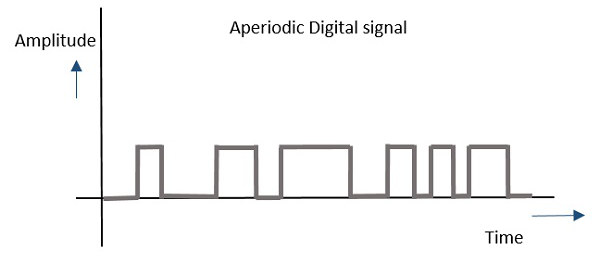In general, the signals which are used in communication systems are analog in nature, which are transmitted in analog or converted to digital and then transmitted, depending upon the requirement.

But for a signal to get transmitted to a distance, without the effect of any external interferences or noise addition and without getting faded away, it has to undergo a process called as Modulation, which is discussed in the next chapter.

# Principles of Communication - Modulation

A signal can be anything like a sound wave which comes out when you shout. This shout can be heard only up to a certain distance. But for the same wave to travel over a long distance, you’ll need a technique which adds strength to this signal, without disturbing the parameters of the original signal.

## What is Signal Modulation?

A message carrying signal has to get transmitted over a distance and for it to establish a reliable communication, it needs to take the help of a high frequency signal which should not affect the original characteristics of the message signal.

The characteristics of the message signal, if changed, the message contained in it also alters. Hence it is a must to take care of the message signal. A high frequency signal can travel up to a longer distance, without getting affected by external disturbances. We take the help of such high frequency signal which is called as a carrier signal to transmit our message signal. Such a process is simply called as Modulation.

Modulation is the process of changing the parameters of the carrier signal, in accordance with the instantaneous values of the modulating signal.

### Need for Modulation

The baseband signals are incompatible for direct transmission. For such a signal, to travel longer distances, its strength has to be increased by modulating with a high frequency carrier wave, which doesn’t affect the parameters of the modulating signal.

The antenna used for transmission, had to be very large, if modulation was not introduced. The range of communication gets limited as the wave cannot travel to a distance without getting distorted.

Following are some of the advantages for implementing modulation in the communication systems.

• Antenna size gets reduced.
• No signal mixing occurs.
• Communication range increases.
• Multiplexing of signals occur.
• Adjustments in the bandwidth is allowed.
• Reception quality improves.

## Signals in the Modulation Process

Following are the three types of signals in the modulation process.

### Message or Modulating Signal

The signal which contains a message to be transmitted, is called as a message signal. It is a baseband signal, which has to undergo the process of modulation, to get transmitted. Hence, it is also called as the modulating signal.

### Carrier Signal

The high frequency signal which has a certain phase, frequency, and amplitude but contains no information, is called a carrier signal. It is an empty signal. It is just used to carry the signal to the receiver after modulation.

### Modulated Signal

The resultant signal after the process of modulation, is called as the modulated signal. This signal is a combination of the modulating signal and the carrier signal.

## Types of Modulation

There are many types of modulations. Depending upon the modulation techniques used, they are classified as shown in the following figure.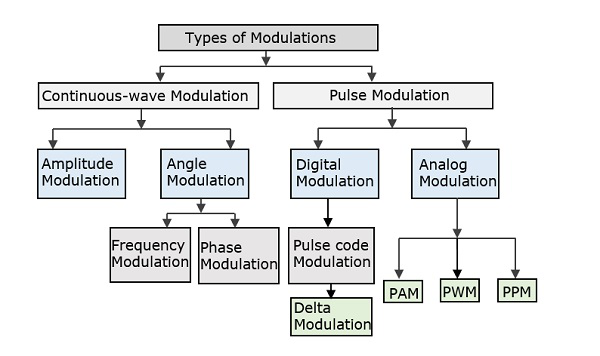The types of modulations are broadly classified into continuous-wave modulation and pulse modulation.

### Continuous-wave Modulation

In the continuous-wave modulation, a high frequency sine wave is used as a carrier wave. This is further divided into amplitude and angle modulation.

• If the amplitude of the high frequency carrier wave is varied in accordance with the instantaneous amplitude of the modulating signal, then such a technique is called as Amplitude Modulation.

• If the angle of the carrier wave is varied, in accordance with the instantaneous value of the modulating signal, then such a technique is called as Angle Modulation.

The angle modulation is further divided into frequency and phase modulation.

• If the frequency of the carrier wave is varied, in accordance with the instantaneous value of the modulating signal, then such a technique is called as Frequency Modulation.

• If the phase of the high frequency carrier wave is varied in accordance with the instantaneous value of the modulating signal, then such a technique is called as Phase Modulation.

### Pulse Modulation

In Pulse modulation, a periodic sequence of rectangular pulses, is used as a carrier wave. This is further divided into analog and digital modulation.

In analog modulation technique, if the amplitude, duration or position of a pulse is varied in accordance with the instantaneous values of the baseband modulating signal, then such a technique is called as Pulse Amplitude Modulation (PAM) or Pulse Duration/Width Modulation (PDM/PWM), or Pulse Position Modulation (PPM).

In digital modulation, the modulation technique used is Pulse Code Modulation (PCM) where the analog signal is converted into digital form of 1s and 0s. As the resultant is a coded pulse train, this is called as PCM. This is further developed as Delta Modulation (DM), which will be discussed in subsequent chapters. Hence, PCM is a technique where the analog signals are converted into a digital form.

# Principles of Communication - Noise

In any communication system, during the transmission of the signal, or while receiving the signal, some unwanted signal gets introduced into the communication, making it unpleasant for the receiver, questioning the quality of the communication. Such a disturbance is called as Noise.

## What is Noise?

Noise is an unwanted signal which interferes with the original message signal and corrupts the parameters of the message signal. This alteration in the communication process, leads to the message getting altered. It is most likely to be entered at the channel or the receiver.

The noise signal can be understood by taking a look at the following example.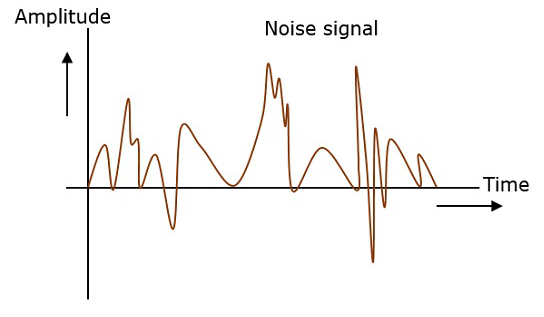Hence, it is understood that noise is some signal which has no pattern and no constant frequency or amplitude. It is quite random and unpredictable. Measures are usually taken to reduce it, though it can’t be completely eliminated.

Most common examples of noise are −

• Buzz sound amidst of telephone conversations

• Flicker in television receivers, etc.

## Effects of Noise

Noise is an inconvenient feature which affects the system performance. Following are the effects of noise.

### Noise limits the operating range of the systems

Noise indirectly places a limit on the weakest signal that can be amplified by an amplifier. The oscillator in the mixer circuit may limit its frequency because of noise. A system’s operation depends on the operation of its circuits. Noise limits the smallest signal that a receiver is capable of processing.

### Noise affects the sensitivity of receivers

Sensitivity is the minimum amount of input signal necessary to obtain the specified quality output. Noise affects the sensitivity of a receiver system, which eventually affects the output.

## Types of Noise

The classification of noise is done depending on the type of the source, the effect it shows or the relation it has with the receiver, etc.

There are two main ways in which noise is produced. One is through some external source while the other is created by an internal source, within the receiver section.

### External Source

This noise is produced by the external sources which may occur in the medium or channel of communication, usually. This noise cannot be completely eliminated. The best way is to avoid the noise from affecting the signal.

### Examples

Most common examples of this type of noise are −

• Atmospheric noise (due to irregularities in the atmosphere).

• Extra-terrestrial noise, such as solar noise and cosmic noise.

• Industrial noise.

### Internal Source

This noise is produced by the receiver components while functioning. The components in the circuits, due to continuous functioning, may produce few types of noise. This noise is quantifiable. A proper receiver design may lower the effect of this internal noise.

### Examples

Most common examples of this type of noise are −

• Thermal agitation noise (Johnson noise or Electrical noise).

• Shot noise (due to the random movement of electrons and holes).

• Transit-time noise (during transition).

• Miscellaneous noise is another type of noise which includes flicker, resistance effect and mixer generated noise, etc.

## Signal to Noise Ratio

Signal-to-Noise Ratio (SNR) is the ratio of the signal power to the noise power. The higher the value of SNR, the greater will be the quality of the received output.

Signal-to-noise ratio at different points can be calculated by using the following formulae −

$$Input \: SNR = (SNR)_I = \frac{Average \: power \: of \: modulating \: signal}{Average \: power \: of \: noise \: at \: input}$$

$$Output \: SNR = (SNR)_O = \frac{Average \: power \: of \: demodulated \: signal}{Average \: power \: of \: noise \: at \: output}$$

$$Channel \: SNR = (SNR)_C = \frac{Average \: power \: of \: modulated \: signal}{Average \: power \: of \: noise \: in \: message \: bandwidth}$$

## Figure of Merit

The ratio of output SNR to the input SNR can be termed as the Figure of merit (F). It is denoted by F. It describes the performance of a device.

$$F = \frac{(SNR)_O}{(SNR)_I}$$

Figure of merit of a receiver is −

$$F = \frac{(SNR)_O}{(SNR)_C}$$

It is so because for a receiver, the channel is the input.

# Analyzing Signals

To analyze a signal, it has to be represented. This representation in communication systems is of two types −

• Frequency domain representation, and
• Time domain representation.

Consider two signals with 1 kHz and 2 kHz frequencies. Both of them are represented in time and frequency domain as shown in the following figure.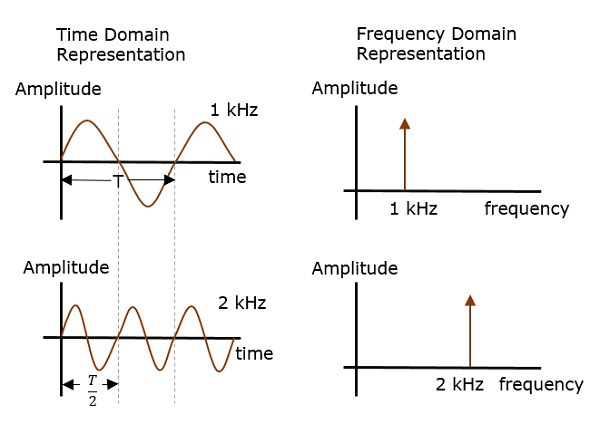Time domain analysis, gives the signal behavior over a certain time period. In the frequency domain, the signal is analyzed as a mathematical function with respect to the frequency.

Frequency domain representation is needed where the signal processing such as filtering, amplifying and mixing are done.

For instance, if a signal such as the following is considered, it is understood that noise is present in it.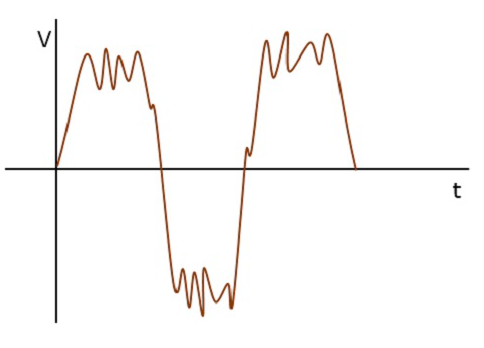The frequency of the original signal may be 1 kHz, but the noise of certain frequency, which corrupts this signal is unknown. However, when the same signal is represented in the frequency domain, using a spectrum analyzer, it is plotted as shown in the following figure.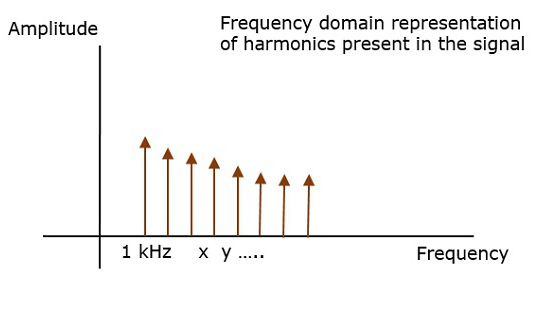Here, we can observe few harmonics, which represent the noise introduced into the original signal. Hence, the signal representation helps in analyzing the signals.

Frequency domain analysis helps in creating the desired wave patterns. For example, the binary bit patterns in a computer, the Lissajous patterns in a CRO, etc. Time domain analysis helps to understand such bit patterns.

# Amplitude Modulation

Among the types of modulation techniques, the main classification is Continuous-wave Modulation and Pulse Modulation. The continuous wave modulation techniques are further divided into Amplitude Modulation and Angle Modulation.

A continuous-wave goes on continuously without any intervals and it is the baseband message signal, which contains the information. This wave has to be modulated.

According to the standard definition, “The amplitude of the carrier signal varies in accordance with the instantaneous amplitude of the modulating signal.” Which means, the amplitude of the carrier signal which contains no information varies as per the amplitude of the signal, at each instant, which contains information. This can be well explained by the following figures.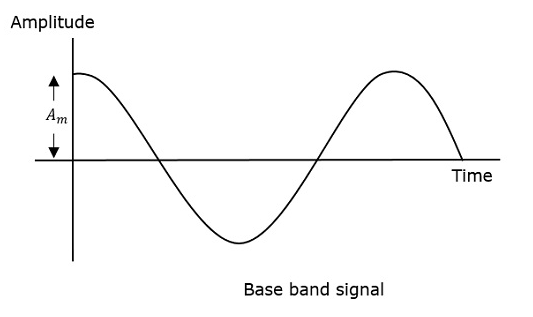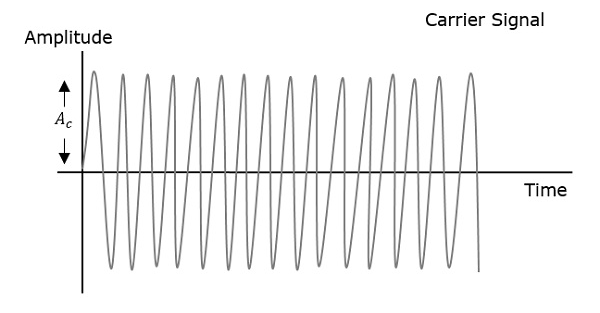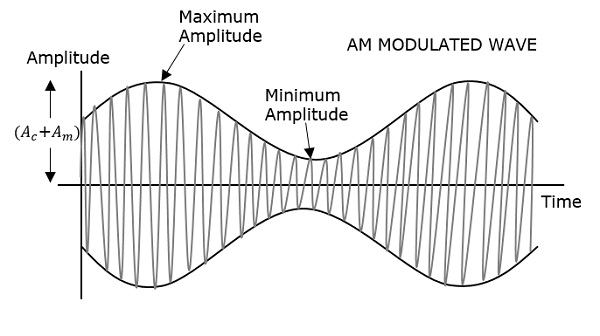The modulating wave which is shown first is the message signal. The next one is the carrier wave, which is just a high frequency signal and contains no information. While the last one is the resultant modulated wave.

It can be observed that the positive and negative peaks of the carrier wave, are interconnected with an imaginary line. This line helps recreating the exact shape of the modulating signal. This imaginary line on the carrier wave is called as Envelope. It is the same as the message signal.

## Mathematical Expression

Following are the mathematical expression for these waves.

### Time-domain Representation of the Waves

Let modulating signal be −

$$m(t) = A_mcos(2\pi f_mt)$$

Let carrier signal be −

$$c(t) = A_ccos(2\pi f_ct)$$

Where Am = maximum amplitude of the modulating signal

Ac = maximum amplitude of the carrier signal

The standard form of an Amplitude Modulated wave is defined as −

$$S(t) = A_c[1+K_am(t)]cos(2\pi f_ct)$$

$$S(t) = A_c[1+\mu cos(2\pi f_mt)]cos(2\pi f_ct)$$

$$Where,\mu = K_aA_m$$

## Modulation Index

A carrier wave, after being modulated, if the modulated level is calculated, then such an attempt is called as Modulation Index or Modulation Depth. It states the level of modulation that a carrier wave undergoes.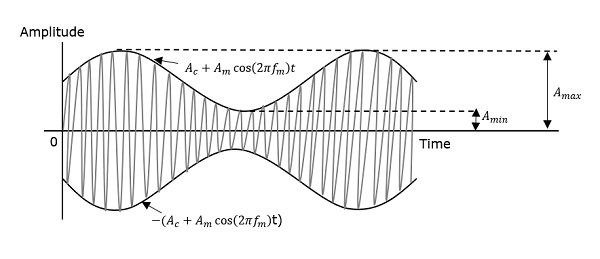The maximum and minimum values of the envelope of the modulated wave are represented by Amax and Amin respectively.

Let us try to develop an equation for the Modulation Index.

$$A_{max} = A_c(1+\mu )$$

Since, at Amax the value of cos θ is 1

$$A_{min} = A_c(1-\mu )$$

Since, at Amin the value of cos θ is -1

$$\frac{A_{max}}{A_{min}} = \frac{1+\mu }{1-\mu }$$

$$A_{max}-\mu A_{max} = A_{min}+\mu A_{min}$$

$$-\mu (A_{max}+A_{min}) = A_{min}-A_{max}$$

$$\mu = \frac{A_{max}-A_{min}}{A_{max}+A_{min}}$$

Hence, the equation for Modulation Index is obtained. µ denotes the modulation index or modulation depth. This is often denoted in percentage called as Percentage Modulation. It is the extent of modulation denoted in percentage, and is denoted by m.

For a perfect modulation, the value of modulation index should be 1, which means the modulation depth should be 100%.

For instance, if this value is less than 1, i.e., the modulation index is 0.5, then the modulated output would look like the following figure. It is called as Under-modulation. Such a wave is called as an under-modulated wave.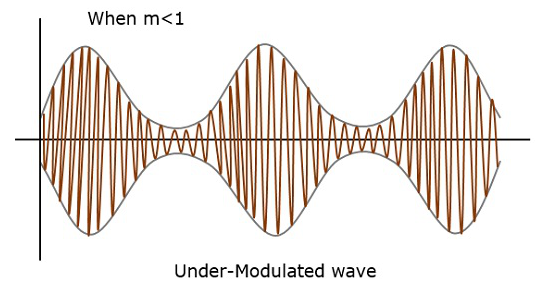If the value of the modulation index is greater than 1, i.e., 1.5 or so, then the wave will be an over-modulated wave. It would look like the following figure.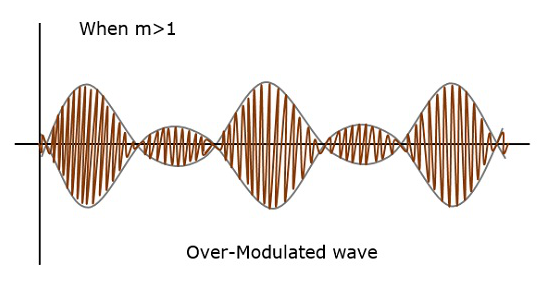As the value of modulation index increases, the carrier experiences a 180° phase reversal, which causes additional sidebands and hence, the wave gets distorted. Such overmodulated wave causes interference, which cannot be eliminated.

## Bandwidth of Amplitude Modulation

The bandwidth is the difference between lowest and highest frequencies of the signal.

For amplitude modulated wave, the bandwidth is given by

$$BW = f_{USB}-f_{LSB}$$

$$(f_c+f_m)-(f_c-f_m)$$

$$= 2f_m = 2W$$

Where W is the message bandwidth

Hence we got to know that the bandwidth required for the amplitude modulated wave is twice the frequency of the modulating signal.

# Sideband Modulation

In the process of Amplitude Modulation or Phase Modulation, the modulated wave consists of the carrier wave and two sidebands. The modulated signal has the information in the whole band except at the carrier frequency.

## Sideband

A Sideband is a band of frequencies, containing power, which are the lower and higher frequencies of the carrier frequency. Both the sidebands contain the same information. The representation of amplitude modulated wave in the frequency domain is as shown in the following figure.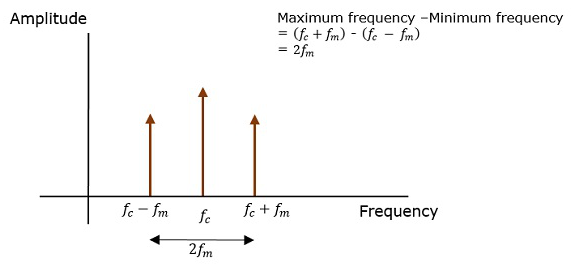Both the sidebands in the image contain the same information. The transmission of such a signal which contains a carrier along with two sidebands, can be termed as Double Sideband Full Carrier system, or simply DSB-FC. It is plotted as shown in the following figure.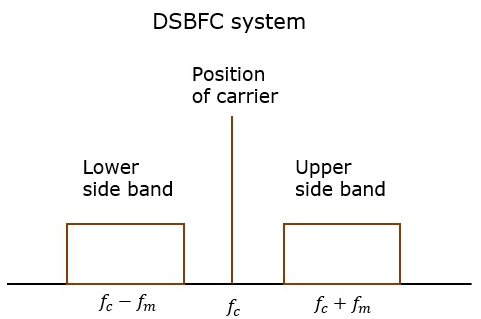However, such a transmission is inefficient. Two-thirds of the power is being wasted in the carrier, which carries no information.

If this carrier is suppressed and the power saved is distributed to the two sidebands, such a process is called as Double Sideband Suppressed Carrier system, or simply DSBSC. It is plotted as shown in the following figure.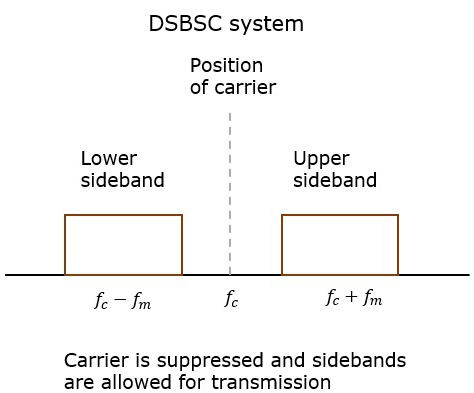Now, we get an idea that, as the two sidebands carry the same information twice, why can’t we suppress one sideband. Yes, this is possible.

The process of suppressing one of the sidebands, along with the carrier and transmitting a single sideband is called as Single Sideband Suppressed Carrier system, or simply SSB-SC or SSB. It is plotted as shown in the following figure.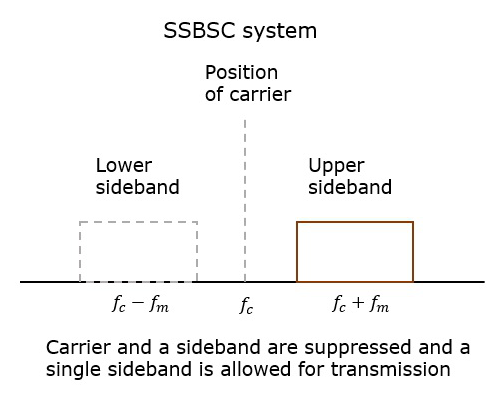This SSB-SC or SSB system, which transmits a single sideband has high power, as the power allotted for both the carrier and the other sideband is utilized in transmitting this Single Sideband (SSB).

Hence, the modulation done using this SSB technique is called as SSB Modulation.

The advantages of SSB modulation are −

• Bandwidth or spectrum space occupied is lesser than AM and DSB signals.

• Transmission of more number of signals is allowed.

• Power is saved.

• High power signal can be transmitted.

• Less amount of noise is present.

• Signal fading is less likely to occur.

The disadvantages of SSB modulation are −

• The generation and detection of SSB signal is a complex process.

• Quality of the signal gets affected unless the SSB transmitter and receiver have an excellent frequency stability.

## Sideband Modulation − Applications

The applications of SSB modulation are −

• For power saving requirements and low bandwidth requirements.

• In land, air, and maritime mobile communications.

• In point-to-point communications.

• In television, telemetry, and radar communications.

• In military communications, such as amateur radio, etc.

# VSB Modulation

In case of SSB modulation, when a sideband is passed through the filters, the band pass filter may not work perfectly in practice. As a result of which, some of the information may get lost.

Hence to avoid this loss, a technique is chosen, which is a compromise between DSB-SC and SSB, called as Vestigial Sideband (VSB) technique. The word vestige which means “a part” from which the name is derived.

## Vestigial Sideband

Both of the sidebands are not required for the transmission, as it is a waste. But a single band if transmitted, leads to loss of information. Hence, this technique has evolved.

Vestigial Sideband Modulation or VSB Modulation is the process where a part of the signal called as vestige is modulated, along with one sideband. A VSB signal can be plotted as shown in the following figure.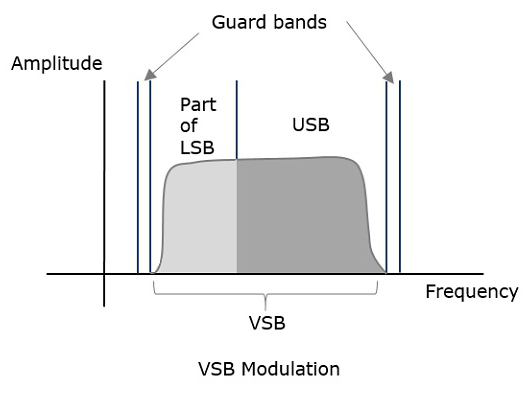Along with the upper sideband, a part of the lower sideband is also being transmitted in this technique. A guard band of very small width is laid on either side of VSB in order to avoid the interferences. VSB modulation is mostly used in television transmissions.

## Transmission Bandwidth

The transmission bandwidth of VSB modulated wave is represented as −

$$B=( f_{m}+ f_{v}) Hz$$

Where,

fm = Message bandwidth

fv = Width of the vestigial sideband

Following are the advantages of VSB −

• Highly efficient.

• Reduction in bandwidth.

• Filter design is easy as high accuracy is not needed.

• The transmission of low frequency components is possible, without difficulty.

• Possesses good phase characteristics.

Following are the disadvantages of VSB −

• Bandwidth when compared to SSB is greater.

• Demodulation is complex.

## VSB Modulation − Application

The most prominent and standard application of VSB is for the transmission of television signals. Also, this is the most convenient and efficient technique when bandwidth usage is considered.

# Angle Modulation

The other type of modulation in continuous-wave modulation is the Angle Modulation. Angle Modulation is the process in which the frequency or the phase of the carrier varies according to the message signal. This is further divided into frequency and phase modulation.

• Frequency Modulation is the process of varying the frequency of the carrier signal linearly with the message signal.

• Phase Modulation is the process of varying the phase of the carrier signal linearly with the message signal.

Let us now discuss these topics in greater detail.

## Frequency Modulation

In amplitude modulation, the amplitude of the carrier varies. But in Frequency Modulation (FM), the frequency of the carrier signal varies in accordance with the instantaneous amplitude of the modulating signal.

The amplitude and the phase of the carrier signal remains constant whereas the frequency of the carrier changes. This can be better understood by observing the following figures.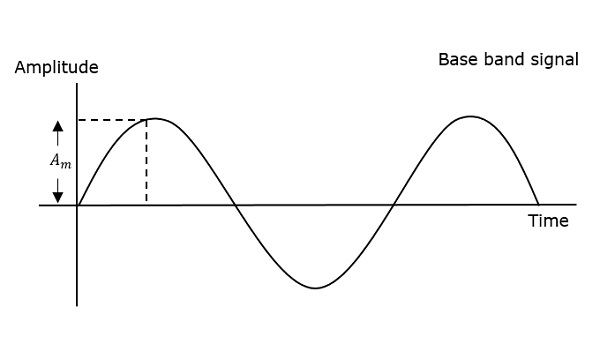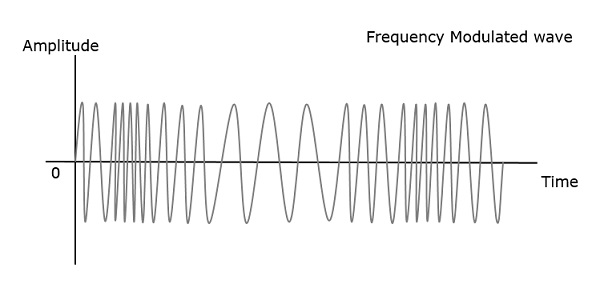The frequency of the modulated wave remains constant as the carrier wave frequency when the message signal is at zero. The frequency increases when the message signal reaches its maximum amplitude.

Which means, with the increase in amplitude of the modulating or message signal, the carrier frequency increases. Likewise, with the decrease in the amplitude of the modulating signal, the frequency also decreases.

### Mathematical Representation

Let the carrier frequency be fc

The frequency at maximum amplitude of the message signal = fc + Δf

The frequency at minimum amplitude of the message signal = fcΔf

The difference between FM modulated frequency and normal frequency is termed as Frequency Deviation and is denoted by Δf.

The deviation of the frequency of the carrier signal from high to low or low to high can be termed as the Carrier Swing.

Carrier Swing = 2 × frequency deviation

= 2 × Δf

### Equation for FM WAVE

The equation for FM wave is −

$$s(t) = A_ccos[W_ct + 2\pi k_fm(t)]$$

Where,

Ac = the amplitude of the carrier

wc = angular frequency of the carrier = 2πfc

m(t) = message signal

FM can be divided into Narrowband FM and Wideband FM.

### Narrowband FM

The features of Narrowband FM are as follows −

• This frequency modulation has a small bandwidth.

• The modulation index is small.

• Its spectrum consists of carrier, USB, and LSB.

• This is used in mobile communications such as police wireless, ambulances, taxicabs, etc.

### Wideband FM

The features of Wideband FM are as follows −

• This frequency modulation has infinite bandwidth.

• The modulation index is large, i.e., higher than 1.

• Its spectrum consists of a carrier and infinite number of sidebands, which are located around it.

• This is used in entertainment broadcasting applications such as FM radio, TV, etc.

## Phase Modulation

In frequency modulation, the frequency of the carrier varies. But in Phase Modulation (PM), the phase of the carrier signal varies in accordance with the instantaneous amplitude of the modulating signal.

The amplitude and the frequency of the carrier signal remains constant whereas the phase of the carrier changes. This can be better understood by observing the following figures.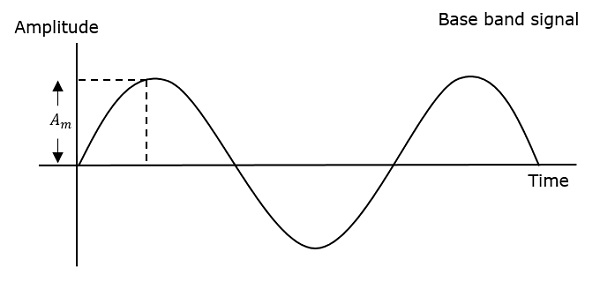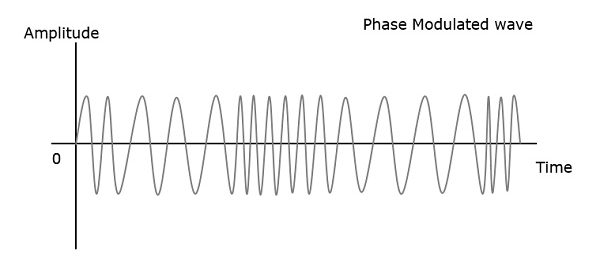The phase of the modulated wave has got infinite points where the phase shift in a wave can take place. The instantaneous amplitude of the modulating signal, changes the phase of the carrier. When the amplitude is positive, the phase changes in one direction and if the amplitude is negative, the phase changes in the opposite direction.

## Relation between PM and FM

The change in phase, changes the frequency of the modulated wave. The frequency of the wave also changes the phase of the wave. Though they are related, their relationship is not linear. Phase modulation is an indirect method of producing FM. The amount of frequency shift, produced by a phase modulator increases with the modulating frequency. An audio equalizer is employed to compensate this.

### Equation for PM Wave

The equation for PM wave is −

$$s(t) = A_ccos[W_ct + k_pm(t)]$$

Where,

Ac = the amplitude of the carrier

wc = angular frequency of the carrier = 2πfc

m(t) = message signal

Phase modulation is used in mobile communication systems, while frequency modulation is used mainly for FM broadcasting.

# Principles of Communication - Multiplexing

Multiplexing is the process of combining multiple signals into one signal, over a shared medium.

• The process is called as analog multiplexing if these signals are analog in nature.

• If digital signals are multiplexed, it is called as digital multiplexing.

Multiplexing was first developed in telephony. A number of signals were combined to send through a single cable. The process of multiplexing divides a communication channel into several number of logical channels, allotting each one for a different message signal or a data stream to be transferred. The device that does multiplexing, can be called as a MUX.

The reverse process, i.e., extracting the number of channels from one, which is done at the receiver is called as demultiplexing. The device which does demultiplexing is called as DEMUX.

The following figures illustrates the concept of MUX and DEMUX. Their primary use is in the field of communications.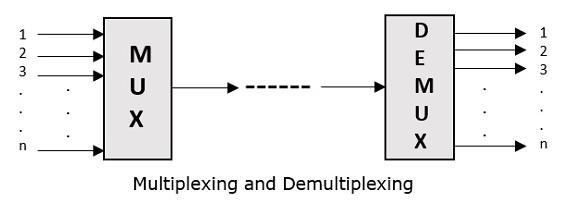## Types of Multiplexers

There are mainly two types of multiplexers, namely analog and digital. They are further divided into FDM, WDM, and TDM. The following figure gives a detailed idea about this classification.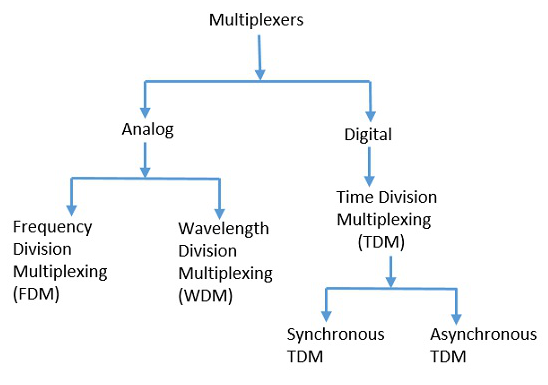There are many types of multiplexing techniques. Of them all, we have the main types with general classification, mentioned in the above figure. Let us take a look at them individually.

## Analog Multiplexing

The analog multiplexing techniques involve signals which are analog in nature. The analog signals are multiplexed according to their frequency (FDM) or wavelength (WDM).

### Frequency Division Multiplexing

In analog multiplexing, the most used technique is Frequency Division Multiplexing (FDM). This technique uses various frequencies to combine streams of data, for sending them on a communication medium, as a single signal.

Example − A traditional television transmitter, which sends a number of channels through a single cable uses FDM.

### Wavelength Division Multiplexing

Wavelength Division multiplexing (WDM) is an analog technique, in which many data streams of different wavelengths are transmitted in the light spectrum. If the wavelength increases, the frequency of the signal decreases. A prism which can turn different wavelengths into a single line, can be used at the output of MUX and input of DEMUX.

Example − Optical fiber Communications use the WDM technique, to merge different wavelengths into a single light for the communication.

## Digital Multiplexing

The term digital represents the discrete bits of information. Hence, the available data is in the form of frames or packets, which are discrete.

### Time Division Multiplexing (TDM)

In TDM, the time frame is divided into slots. This technique is used to transmit a signal over a single communication channel, by allotting one slot for each message.

Of all the types of TDM, the main ones are Synchronous and Asynchronous TDM.

### Synchronous TDM

In Synchronous TDM, the input is connected to a frame. If there are ‘n’ number of connections, then the frame is divided into ‘n’ time slots. One slot is allocated for each input line.

In this technique, the sampling rate is common for all signals and hence the same clock input is given. The MUX allocates the same slot to each device at all times.

### Asynchronous TDM

In Asynchronous TDM, the sampling rate is different for each of the signals and a common clock is not required. If the allotted device, for a time slot transmits nothing and sits idle, then that slot is allotted to another device, unlike synchronous.

This type of TDM is used in Asynchronous transfer mode networks.

## Demultiplexer

Demultiplexers are used to connect a single source to multiple destinations. This process is the reverse of multiplexing. As mentioned previously, it is used mostly at the receivers. DEMUX has many applications. It is used in receivers in the communication systems. It is used in arithmetic and logical unit in computers to supply power and to pass on communication, etc.

Demultiplexers are used as serial to parallel converters. The serial data is given as input to DEMUX at regular interval and a counter is attached to it to control the output of the demultiplexer.

Both the multiplexers and demultiplexers play an important role in communication systems, both at the transmitter and receiver sections.

# Principles of Communication - FM Radio

Frequency division multiplexing is used in radio and television receivers. The main use of FM is for radio communications. Let us take a look at the structure of FM transmitter and FM receiver along with their block diagrams and working.

## FM Transmitter

FM transmitter is the whole unit which takes the audio signal as an input and delivers FM modulated waves to the antenna as an output to be transmitted. FM transmitter consists of 6 main stages. They are illustrated in the following figure.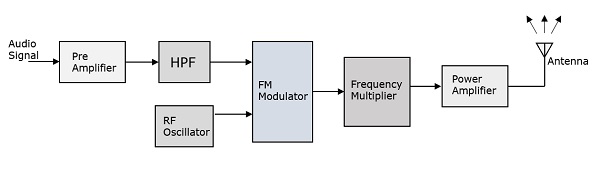The working of FM transmitter can be explained as follows.

• The audio signal from the output of the microphone is given to the pre-amplifier which boosts the level of the modulating signal.

• This signal is then passed to the high pass filter, which acts as a pre-emphasis network to filter out the noise and improve the signal to noise ratio.

• This signal is further passed to the FM modulator circuit.

• The oscillator circuit generates a high frequency carrier, which is given to the modulator along with the modulating signal.

• Several stages of frequency multiplier are used to increase the operating frequency. Even then, the power of the signal is not enough to transmit. Hence, a RF power amplifier is used at the end to increase the power of the modulated signal. This FM modulated output is finally passed to the antenna to get transmitted.

A radio receiver is used to receive both AM band and FM band signals. The detection of AM is done by the method called as Envelope Detection and the detection of FM is done by the method called as Frequency Discrimination.

• It should be cost effective.

• It should receive both AM and FM signals.

• The receiver should be able to tune and amplify the desired station.

• It should have an ability to reject the unwanted stations.

• Demodulation has to be done to all the station signals, whatever the carrier frequency is.

For these requirements to get fulfilled, the tuner circuit and the mixer circuit should be very effective. The procedure of RF mixing is an interesting phenomenon.

## RF Mixing

The RF mixing unit develops an Intermediate Frequency (IF) to which any received signal is converted, so as to process the signal effectively.

RF Mixer is an important stage in the receiver. Two signals of different frequencies are taken where one signal level affects the level of the other signal, to produce the resultant mixed output. The input signals and the resultant mixer output is illustrated in the following figures.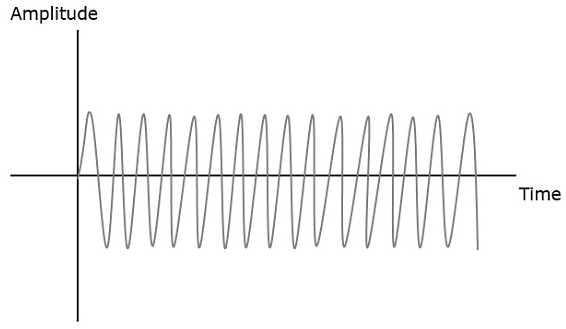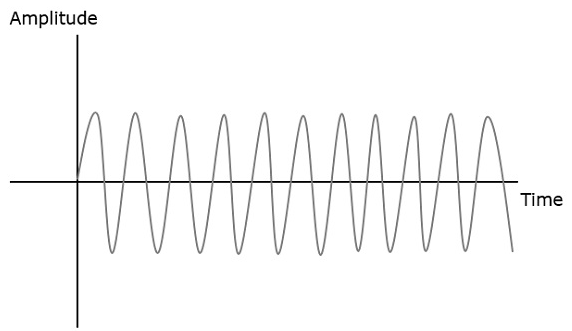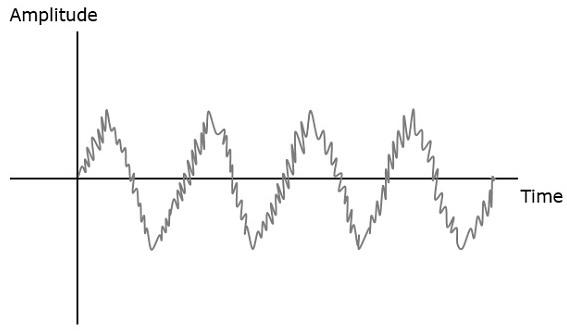When two signals enter the RF mixer,

• The first signal frequency = F1

• The second signal frequency = F2

Then, the resultant signal frequencies = (F1 + F2) and (F1 - F2)

A mixer of two signals of different frequencies are produced at the output.

If this is observed in frequency domain, the pattern looks like the following figure.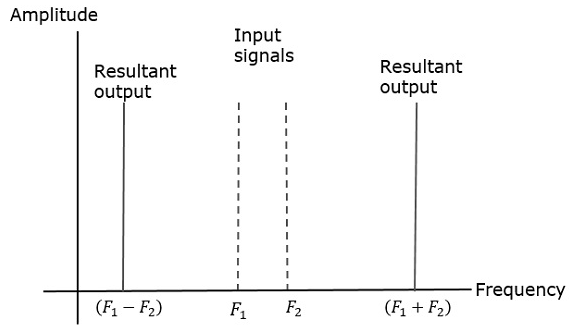The symbol of a RF mixer looks like the following figure.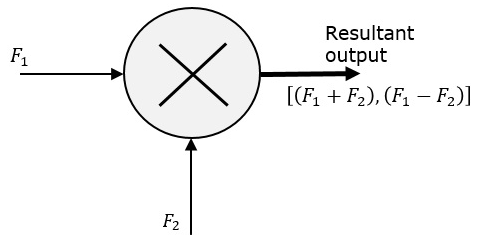The two signals are mixed to produce a resultant signal, where the effect of one signal, affects the other signal and both produce a different pattern as seen previously.

The FM receiver is the whole unit which takes the modulated signal as input and produces the original audio signal as an output. Radio amateurs are the initial radio receivers. However, they have drawbacks such as poor sensitivity and selectivity.

Selectivity is the selection of a particular signal while rejecting the others. Sensitivity is the capacity of detecting a RF signal and demodulating it, while at the lowest power level.

To overcome these drawbacks, super heterodyne receiver was invented. This FM receiver consists of 5 main stages. They are as shown in the following figure.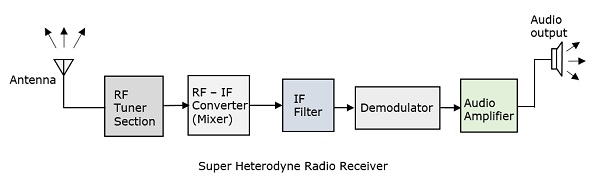### RF Tuner Section

The modulated signal received by the antenna is first passed to the tuner circuit through a transformer. The tuner circuit is nothing but a LC circuit, which is also called as resonant or tank circuit. It selects the frequency, desired by the radio receiver. It also tunes the local oscillator and the RF filter at the same time.

### RF Mixer

The signal from the tuner output is given to the RF-IF converter, which acts as a mixer. It has a local oscillator, which produces a constant frequency. The mixing process is done here, having the received signal as one input and the local oscillator frequency as the other input. The resultant output is a mixture of two frequencies [(f1 + f2),(f1 − f2)] produced by the mixer, which is called as the Intermediate Frequency (IF).

The production of IF helps in the demodulation of any station signal having any carrier frequency. Hence, all signals are translated to a fixed carrier frequency for adequate selectivity.

### IF Filter

Intermediate frequency filter is a bandpass filter, which passes the desired frequency. It eliminates any unwanted higher frequency components present in it as well as the noise. IF filter helps in improving the Signal to Noise Ratio (SNR).

### Demodulator

The received modulated signal is now demodulated with the same process used at the transmitter side. The frequency discrimination is generally used for FM detection.

### Audio Amplifier

This is the power amplifier stage which is used to amplify the detected audio signal. The processed signal is given strength to be effective. This signal is passed on to the loudspeaker to get the original sound signal.

This super heterodyne receiver is well used because of its advantages such as better SNR, sensitivity and selectivity.

## Noise in FM

The presence of noise is a problem in FM as well. Whenever a strong interference signal with closer frequency to the desired signal arrives, the receiver locks that interference signal. Such a phenomenon is called as the Capture effect.

To increase the SNR at higher modulation frequencies, a high pass circuit called preemphasis, is used at the transmitter. Another circuit called de-emphasis, the inverse process of pre-emphasis is used at the receiver, which is a low pass circuit. The preemphasis and de-emphasis circuits are widely used in FM transmitter and receiver to effectively increase the output SNR.

# Pulse Modulation

So far, we have discussed about continuous-wave modulation. Now it’s time for discrete signals. The Pulse modulation techniques, deals with discrete signals. Let us see how to convert a continuous signal into a discrete one. The process called Sampling helps us with this.

## Sampling

The process of converting continuous time signals into equivalent discrete time signals, can be termed as Sampling. A certain instant of data is continually sampled in the sampling process.

The following figure indicates a continuous-time signal x(t) and a sampled signal xs(t). When x(t) is multiplied by a periodic impulse train, the sampled signal xs(t) is obtained.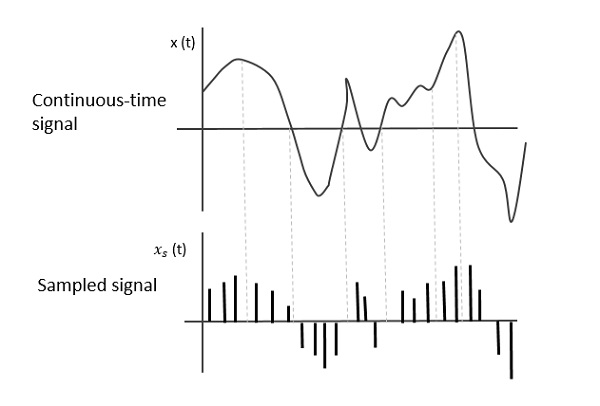A sampling signal is a periodic train of pulses, having unit amplitude, sampled at equal intervals of time Ts, which is called as the Sampling time. This data is transmitted at the time instants Ts and the carrier signal is transmitted at the remaining time.

## Sampling Rate

To discretize the signals, the gap between the samples should be fixed. That gap can be termed as the sampling period Ts.

$$Sampling\:Frequency = \frac{1}{T_s} = f_s$$

Where,

Ts = the sampling time

fs = the sampling frequency or sampling rate

## Sampling Theorem

While considering the sampling rate, an important point regarding how much the rate has to be, should be considered. The rate of sampling should be such that the data in the message signal should neither be lost nor it should get over-lapped.

The sampling theorem states that, “a signal can be exactly reproduced if it is sampled at the rate fs which is greater than or equal to twice the maximum frequency W.”

To put it in simpler words, for the effective reproduction of the original signal, the sampling rate should be twice the highest frequency.

Which means,

$$f_s \geq 2W$$

Where,

fs = the sampling frequency

W is the highest frequency

This rate of sampling is called as Nyquist rate.

The sampling theorem, which is also called as Nyquist theorem, delivers the theory of sufficient sample rate in terms of bandwidth for the class of functions that are bandlimited.

For the continuous-time signal x(t), the band-limited signal in frequency domain, can be represented as shown in the following figure.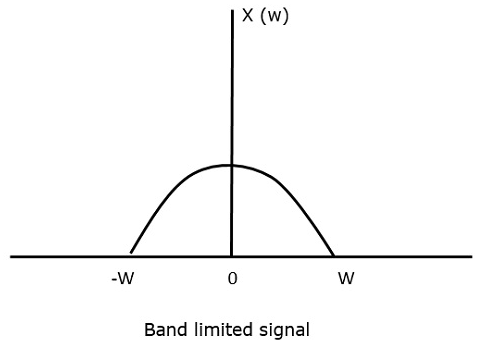If the signal is sampled above the Nyquist rate, the original signal can be recovered. The following figure explains a signal, if sampled at a higher rate than 2w in the frequency domain.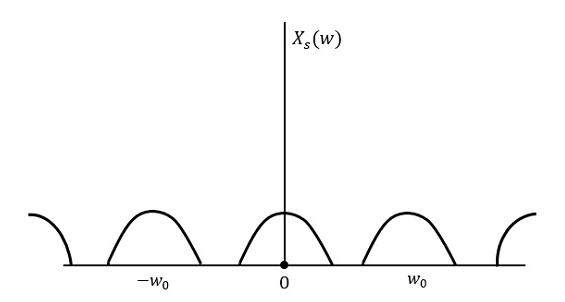If the same signal is sampled at a rate less than 2w, then the sampled signal would look like the following figure.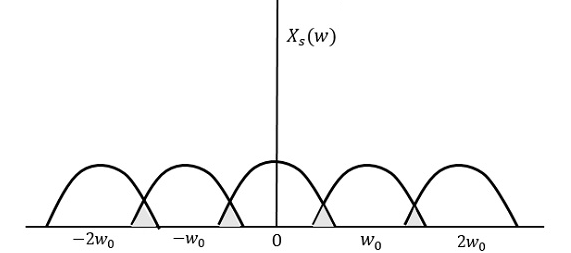We can observe from the above pattern that the over-lapping of information is done, which leads to mixing up and loss of information. This unwanted phenomenon of over-lapping is called as Aliasing.

Aliasing can be referred to as “the phenomenon of a high-frequency component in the spectrum of a signal, taking on the identity of a lower-frequency component in the spectrum of its sampled version.”

Hence, the sampling of the signal is chosen to be at the Nyquist rate, as was stated in the sampling theorem. If the sampling rate is equal to twice the highest frequency (2W).

That means,

$$f_s = 2W$$

Where,

fs = the sampling frequency

W is the highest frequency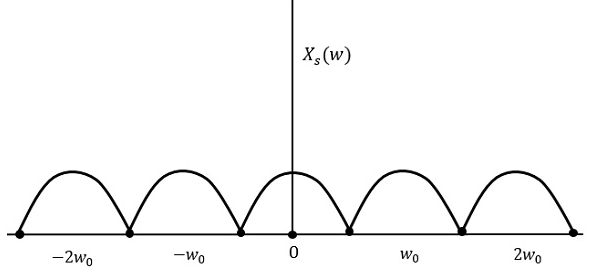The result will be as shown in the above figure. The information is replaced without any loss. Hence, this is a good sampling rate.

# Analog Pulse Modulation

After the continuous wave modulation, the next division is Pulse modulation. Pulse modulation is further divided into analog and digital modulation. The analog modulation techniques are mainly classified into Pulse Amplitude Modulation, Pulse Duration Modulation/Pulse Width Modulation, and Pulse Position Modulation.

## Pulse Amplitude Modulation

Pulse Amplitude Modulation (PAM) is an analog modulating scheme in which the amplitude of the pulse carrier varies proportional to the instantaneous amplitude of the message signal.

The pulse amplitude modulated signal, will follow the amplitude of the original signal, as the signal traces out the path of the whole wave. In natural PAM, a signal sampled at the Nyquist rate is reconstructed, by passing it through an efficient Low Pass Frequency (LPF) with exact cutoff frequency

The following figures explain the Pulse Amplitude Modulation.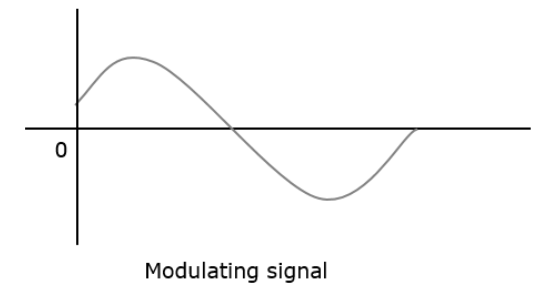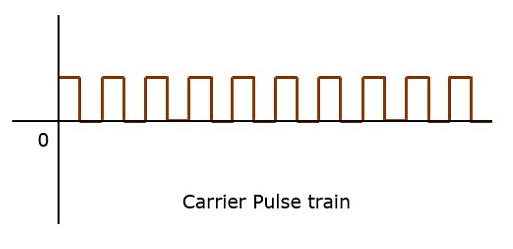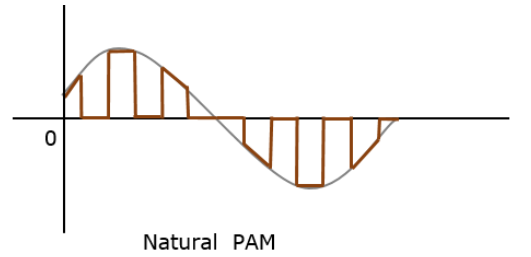Though the PAM signal is passed through an LPF, it cannot recover the signal without distortion. Hence to avoid this noise, flat-top sampling is done as shown in the following figure.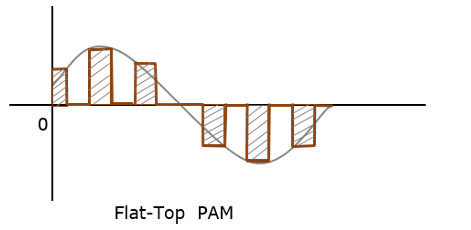Flat-top sampling is the process in which sampled signal can be represented in pulses for which the amplitude of the signal cannot be changed with respect to the analog signal, to be sampled. The tops of amplitude remain flat. This process simplifies the circuit design.

## Pulse Width Modulation

Pulse Width Modulation (PWM) or Pulse Duration Modulation (PDM) or Pulse Time Modulation (PTM) is an analog modulating scheme in which the duration or width or time of the pulse carrier varies proportional to the instantaneous amplitude of the message signal.

The width of the pulse varies in this method, but the amplitude of the signal remains constant. Amplitude limiters are used to make the amplitude of the signal constant. These circuits clip off the amplitude, to a desired level and hence the noise is limited.

The following figures explain the types of Pulse Width Modulations.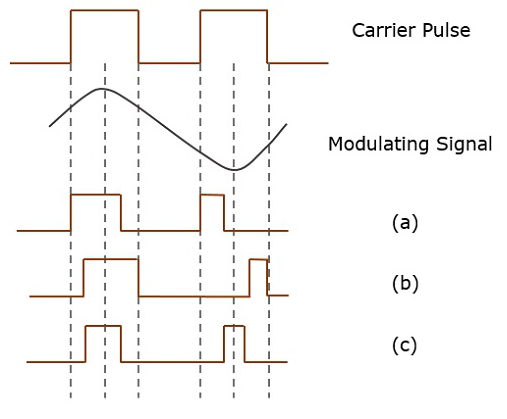There are three variations of PWM. They are −

• The leading edge of the pulse being constant, the trailing edge varies according to the message signal.

• The trailing edge of the pulse being constant, the leading edge varies according to the message signal.

• The center of the pulse being constant, the leading edge and the trailing edge varies according to the message signal.

These three types are shown in the above given figure, with timing slots.

## Pulse Position Modulation

Pulse Position Modulation (PPM) is an analog modulating scheme in which the amplitude and width of the pulses are kept constant, while the position of each pulse, with reference to the position of a reference pulse varies according to the instantaneous sampled value of the message signal.

The transmitter has to send synchronizing pulses (or simply sync pulses) to keep the transmitter and receiver in synchronism. These sync pulses help maintain the position of the pulses. The following figures explain the Pulse Position Modulation.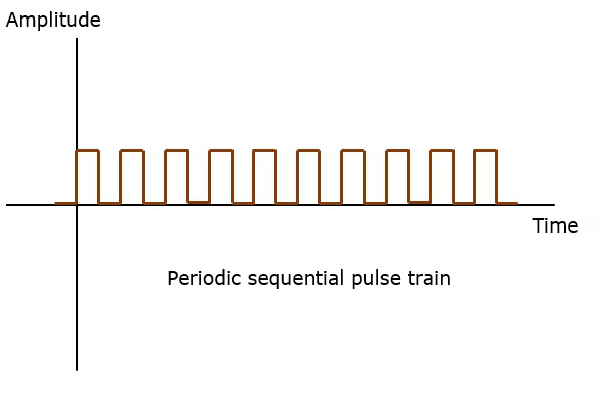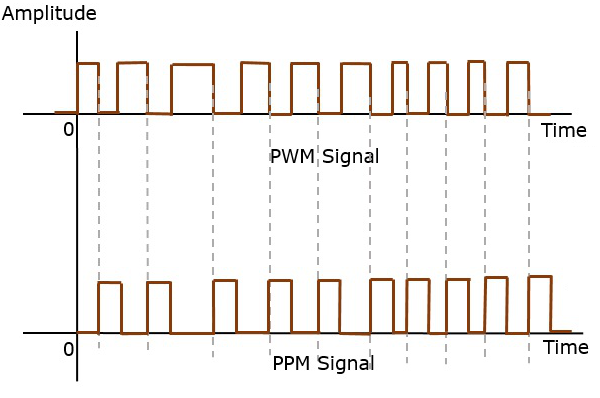Pulse position modulation is done in accordance with the pulse width modulated signal. Each trailing of the pulse width modulated signal becomes the starting point for pulses in PPM signal. Hence, the position of these pulses is proportional to the width of the PWM pulses.

As the amplitude and width are constant, the power handled is also constant.

The synchronization between transmitter and receiver is a must.

## Comparison between PAM, PWM, and PPM

The comparison between the above modulation processes is presented in a single table.

PAM PWM PPM
Amplitude is varied Width is varied Position is varied
Bandwidth depends on the width of the pulse Bandwidth depends on the rise time of the pulse Bandwidth depends on the rise time of the pulse
Instantaneous transmitter power varies with the amplitude of the pulses Instantaneous transmitter power varies with the amplitude and width of the pulses Instantaneous transmitter power remains constant with the width of the pulses
System complexity is high System complexity is low System complexity is low
Noise interference is high Noise interference is low Noise interference is low
It is similar to amplitude modulation It is similar to frequency modulation It is similar to phase modulation

# Digital Modulation

So far we have gone through different modulation techniques. The one remaining is digital modulation, which falls under the classification of pulse modulation. Digital modulation has Pulse Code Modulation (PCM) as the main classification. It further gets processed to delta modulation and ADM.

## Pulse Code Modulation

A signal is Pulse Code modulated to convert its analog information into a binary sequence, i.e., 1s and 0s. The output of a Pulse Code Modulation (PCM) will resemble a binary sequence. The following figure shows an example of PCM output with respect to instantaneous values of a given sine wave.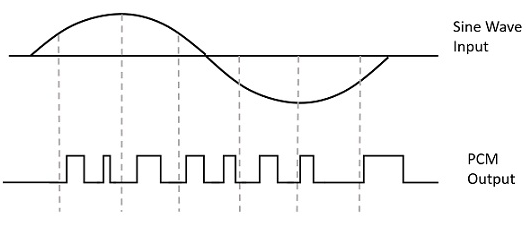Instead of a pulse train, PCM produces a series of numbers or digits, and hence this process is called as digital. Each one of these digits, though in binary code, represent the approximate amplitude of the signal sample at that instant.

In Pulse Code Modulation, the message signal is represented by a sequence of coded pulses. This message signal is achieved by representing the signal in discrete form in both time and amplitude.

## Basic Elements of PCM

The transmitter section of a Pulse Code Modulator circuit consists of Sampling, Quantizing and Encoding, which are performed in the analog-to-digital converter section. The low pass filter prior to sampling prevents aliasing of the message signal.

The basic operations in the receiver section are regeneration of impaired signals, decoding, and reconstruction of the quantized pulse train. The following figure is the block diagram of PCM which represents the basic elements of both the transmitter and the receiver sections.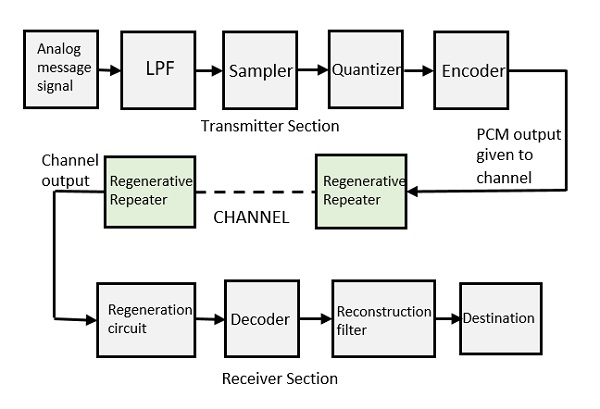### Low Pass Filter (LPF)

This filter eliminates the high frequency components present in the input analog signal which is greater than the highest frequency of the message signal, to avoid aliasing of the message signal.

### Sampler

This is the circuit which uses the technique that helps to collect the sample data at instantaneous values of the message signal, so as to reconstruct the original signal. The sampling rate must be greater than twice the highest frequency component W of the message signal, in accordance with the sampling theorem.

### Quantizer

Quantizing is a process of reducing the excessive bits and confining the data. The sampled output when given to Quantizer, reduces the redundant bits and compresses the value.

### Encoder

The digitization of analog signal is done by the encoder. It designates each quantized level by a binary code. The sampling done here is the sample-and-hold process. These three sections will act as an analog to the digital converter. Encoding minimizes the bandwidth used.

### Regenerative Repeater

The output of the channel has one regenerative repeater circuit to compensate the signal loss and reconstruct the signal. It also increases the strength of the signal.

### Decoder

The decoder circuit decodes the pulse coded waveform to reproduce the original signal. This circuit acts as the demodulator.

### Reconstruction Filter

After the digital-to-analog conversion is done by the regenerative circuit and the decoder, a low pass filter is employed, called as the reconstruction filter to get back the original signal.

Hence, the Pulse Code Modulator circuit digitizes the analog signal given, codes it, and samples it. It then transmits in an analog form. This whole process is repeated in a reverse pattern to obtain the original signal.

# Modulation Techniques

There are few modulation techniques which are followed to construct a PCM signal. These techniques like sampling, quantization, and companding help to create an effective PCM signal, which can exactly reproduce the original signal.

## Quantization

The digitization of analog signals involves the rounding off of the values which are approximately equal to the analog values. The method of sampling chooses few points on the analog signal and then these points are joined to round off the value to a near stabilized value. Such a process is called as Quantization.

The quantizing of an analog signal is done by discretizing the signal with a number of quantization levels. Quantization is representing the sampled values of the amplitude by a finite set of levels, which means converting a continuous-amplitude sample into a discrete-time signal.

The following figure shows how an analog signal gets quantized. The blue line represents analog signal while the red one represents the quantized signal.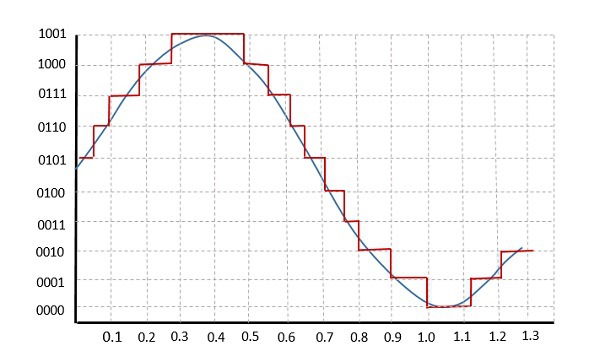Both sampling and quantization results in the loss of information. The quality of a Quantizer output depends upon the number of quantization levels used. The discrete amplitudes of the quantized output are called as representation levels or reconstruction levels. The spacing between two adjacent representation levels is called a quantum or step-size.

## Companding in PCM

The word Companding is a combination of Compressing and Expanding, which means that it does both. This is a non-linear technique used in PCM which compresses the data at the transmitter and expands the same data at the receiver. The effects of noise and crosstalk are reduced by using this technique.

There are two types of Companding techniques.

### A-law Companding Technique

• Uniform quantization is achieved at A = 1, where the characteristic curve is linear and there is no compression.

• A-law has mid-rise at the origin. Hence, it contains a non-zero value.

• A-law companding is used for PCM telephone systems.

• A-law is used in many parts of the world.

### µ-law Companding Technique

• Uniform quantization is achieved at µ = 0, where the characteristic curve is linear and there is no compression.

• µ-law has mid-tread at the origin. Hence, it contains a zero value.

• µ-law companding is used for speech and music signals.

• µ-law is used in North America and Japan.

## Differential PCM

The samples that are highly correlated, when encoded by PCM technique, leave redundant information behind. To process this redundant information and to have a better output, it is a wise decision to take predicted sampled values, assumed from its previous outputs and summarize them with the quantized values.

Such a process is named as Differential PCM technique.

# Delta Modulation

The sampling rate of a signal should be higher than the Nyquist rate, to achieve better sampling. If this sampling interval in a Differential PCM (DPCM) is reduced considerably, the sample-to-sample amplitude difference is very small, as if the difference is 1-bit quantization, then the step-size is very small i.e., Δ (delta).

## What is Delta Modulation?

The type of modulation, where the sampling rate is much higher and in which the stepsize after quantization is of smaller value Δ, such a modulation is termed as delta modulation.

### Features of Delta Modulation

• An over-sampled input is taken to make full use of a signal correlation.

• The quantization design is simple.

• The input sequence is much higher than Nyquist rate.

• The quality is moderate.

• The design of the modulator and the demodulator is simple.

• The stair-case approximation of output waveform.

• The step-size is very small, i.e., Δ (delta).

• The bit rate can be decided by the user.

• It requires simpler implementation.

Delta Modulation is a simplified form of DPCM technique, also viewed as 1-bit DPCM scheme. As the sampling interval is reduced, the signal correlation will be higher.

## Delta Modulator

The Delta Modulator comprises of a 1-bit quantizer and a delay circuit along with two summer circuits. Following is the block diagram of a delta modulator.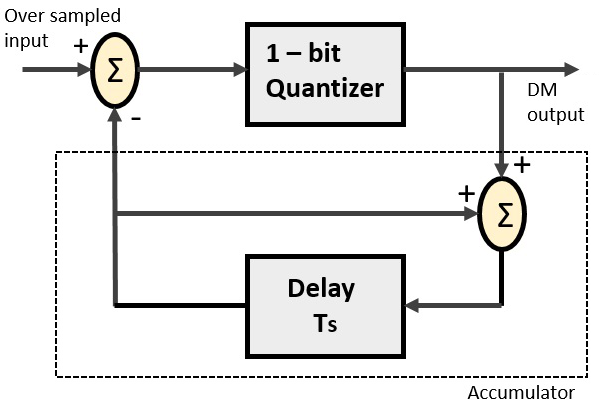A stair-case approximated waveform will be the output of the delta modulator with the step-size as delta (Δ). The output quality of the waveform is moderate.

## Delta Demodulator

The delta demodulator comprises of a low pass filter, a summer, and a delay circuit. The predictor circuit is eliminated here and hence no assumed input is given to the demodulator.

Following is the block diagram for delta demodulator.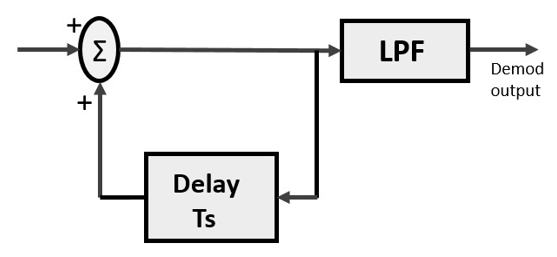Low pass filter is used for many reasons, but the prominent one is noise elimination for out-of-band signals. The step-size error that may occur at the transmitter is called granular noise, which is eliminated here. If there is no noise present, then the modulator output equals the demodulator input.

### Advantages of DM over DPCM

• 1-bit quantizer
• Very easy design of modulator & demodulator

However, there exists some noise in DM and following are the types of noise.

• Slope Over load distortion (when Δ is small)
• Granular noise (when Δ is large)

In digital modulation, we come across certain problems in determining the step-size, which influences the quality of the output wave.

The larger step-size is needed in the steep slope of modulating signal and a smaller stepsize is needed where the message has a small slope. As a result, the minute details get missed. Hence, it would be better if we can control the adjustment of step-size, according to our requirement in order to obtain the sampling in a desired fashion. This is the concept of Adaptive Delta Modulation (ADM).

# Digital Modulation Techniques

Digital Modulation provides more information capacity, high data security, quicker system availability with great quality communication. Hence, digital modulation techniques have a greater demand, for their capacity to convey larger amounts of data than analog ones.

There are many types of digital modulation techniques and we can even use a combination of these techniques as well. In this chapter, we will be discussing the most prominent digital modulation techniques.

## Amplitude Shift Keying

The amplitude of the resultant output depends upon the input data whether it should be a zero level or a variation of positive and negative, depending upon the carrier frequency.

Amplitude Shift Keying (ASK) is a type of Amplitude Modulation which represents the binary data in the form of variations in the amplitude of a signal.

Following is the diagram for ASK modulated waveform along with its input.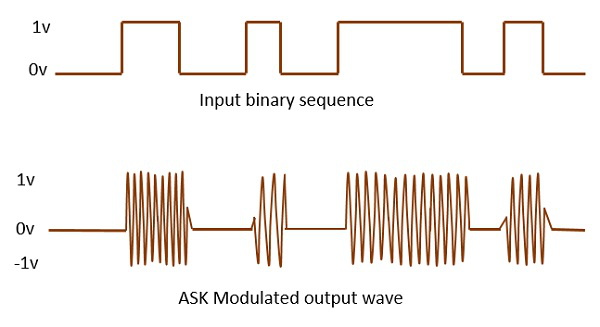Any modulated signal has a high frequency carrier. The binary signal when ASK is modulated, gives a zero value for LOW input and gives the carrier output for HIGH input.

## Frequency Shift Keying

The frequency of the output signal will be either high or low, depending upon the input data applied.

Frequency Shift Keying (FSK) is the digital modulation technique in which the frequency of the carrier signal varies according to the discrete digital changes. FSK is a scheme of frequency modulation.

Following is the diagram for FSK modulated waveform along with its input.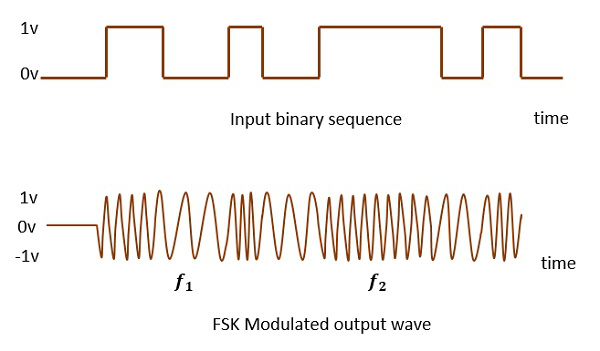The output of a FSK modulated wave is high in frequency for a binary HIGH input and is low in frequency for a binary LOW input. The binary 1s and 0s are called Mark and Space frequencies.

## Phase Shift Keying

The phase of the output signal gets shifted depending upon the input. These are mainly of two types, namely BPSK and QPSK, according to the number of phase shifts. The other one is DPSK which changes the phase according to the previous value.

Phase Shift Keying (PSK) is the digital modulation technique in which the phase of the carrier signal is changed by varying the sine and cosine inputs at a particular time. PSK technique is widely used for wireless LANs, bio-metric, contactless operations, along with RFID and Bluetooth communications.

PSK is of two types, depending upon the phases the signal gets shifted. They are −

### Binary Phase Shift Keying (BPSK)

This is also called as 2-phase PSK (or) Phase Reversal Keying. In this technique, the sine wave carrier takes two phase reversals such as 0° and 180°.

BPSK is basically a DSB-SC (Double Sideband Suppressed Carrier) modulation scheme, for message being the digital information.

Following is the image of BPSK Modulated output wave along with its input.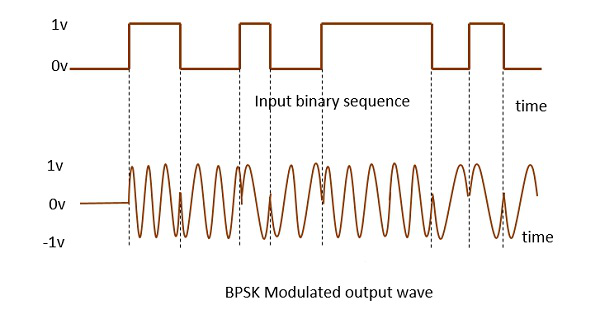### Quadrature Phase Shift Keying (QPSK)

This is the phase shift keying technique, in which the sine wave carrier takes four phase reversals such as 0°, 90°, 180°, and 270°.

If this kind of techniques are further extended, PSK can be done by eight or sixteen values also, depending upon the requirement. The following figure represents the QPSK waveform for two bits input, which shows the modulated result for different instances of binary inputs.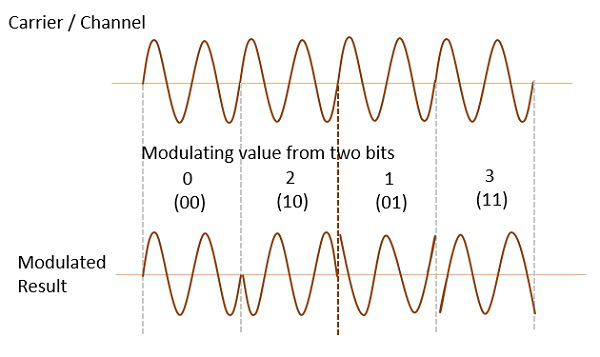QPSK is a variation of BPSK, and it is also a DSB-SC (Double Sideband Suppressed Carrier) modulation scheme, which send two bits of digital information at a time, called as bigits.

Instead of the conversion of digital bits into a series of digital stream, it converts them into bit-pairs. This decreases the data bit rate to half, which allows space for the other users.

### Differential Phase Shift Keying (DPSK)

In DPSK (Differential Phase Shift Keying) the phase of the modulated signal is shifted relative to the previous signal element. No reference signal is considered here. The signal phase follows the high or low state of the previous element. This DPSK technique doesn’t need a reference oscillator.

The following figure represents the model waveform of DPSK.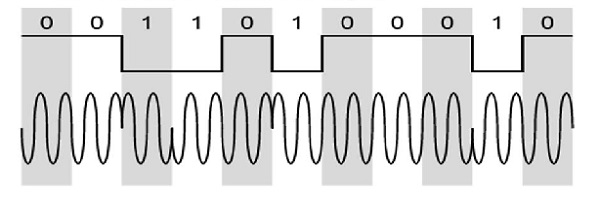It is seen from the above figure that, if the data bit is LOW i.e., 0, then the phase of the signal is not reversed, but is continued as it was. If the data is HIGH i.e., 1, then the phase of the signal is reversed, as with NRZI, invert on 1 (a form of differential encoding).

If we observe the above waveform, we can say that the HIGH state represents an M in the modulating signal and the LOW state represents a W in the modulating signal.

# M-ary Encoding

The word binary represents two-bits. M simply represents a digit that corresponds to the number of conditions, levels, or combinations possible for a given number of binary variables.

This is the type of digital modulation technique used for data transmission in which instead of one-bit, two or more bits are transmitted at a time. As a single signal is used for multiple bit transmission, the channel bandwidth is reduced.

## M-ary Equation

If a digital signal is given under four conditions, such as voltage levels, frequencies, phases and amplitude, then M = 4.

The number of bits necessary to produce a given number of conditions is expressed mathematically as

$$N = \log_{2}M$$

Where,

N is the number of bits necessary.

M is the number of conditions, levels, or combinations possible with N bits.

The above equation can be re-arranged as −

$$2^{N} = M$$

For example, with two bits, 22 = 4 conditions are possible.

## Types of M-ary Techniques

In general, (M-ary) multi-level modulation techniques are used in digital communications as the digital inputs with more than two modulation levels allowed on the transmitter’s input. Hence, these techniques are bandwidth efficient.

There are many different M-ary modulation techniques. Some of these techniques, modulate one parameter of the carrier signal, such as amplitude, phase, and frequency.

This is called M-ary Amplitude Shift Keying (M-ASK) or M-ary Pulse Amplitude Modulation (PAM).

The amplitude of the carrier signal, takes on M different levels.

$$S_m(t) = A_mcos(2\pi f_ct)\:\:\:\:\:\:A_m\epsilon {(2m-1-M)\Delta ,m = 1,2....M}\:\:\:and\:\:\:0\leq t\leq T_s$$

This method is also used in PAM. Its implementation is simple. However, M-ary ASK is susceptible to noise and distortion.

## M-ary FSK

This is called as M-ary Frequency Shift Keying.

The frequency of the carrier signal, takes on M different levels.

### Representation of M-ary FSK

$$S_{i} (t) = \sqrt{\frac{2E_{s}}{T_{S}}} \cos\lgroup\frac{\Pi} {T_{s}}(n_{c} + i)t\rgroup \:\:\:\:0\leq t\leq T_{s}\:\:\:and\:\:\:i = 1,2.....M$$

where $f_{c} = \frac{n_{c}}{2T_{s}}$ for some fixed integer n.

This is not susceptible to noise as much as ASK. The transmitted M number of signals are equal in energy and duration. The signals are separated by $\frac{1}{2T_s}$ Hz making the signals orthogonal to each other.

Since M signals are orthogonal, there is no crowding in the signal space. The bandwidth efficiency of an M-ary FSK decreases and the power efficiency increases with the increase in M.

## M-ary PSK

This is called as M-ary Phase Shift Keying.

The phase of the carrier signal, takes on M different levels.

### Representation of M-ary PSK

$$S_{i}(t) = \sqrt{\frac{2E}{T}} \cos(w_{0}t + \emptyset_{i}t)\:\:\:\:0\leq t\leq T_{s}\:\:\:and\:\:\:i = 1,2.....M$$

$$\emptyset_{i}t = \frac{2\Pi i} {M}\:\:\:where\:\:i = 1,2,3...\:...M$$

Here, the envelope is constant with more phase possibilities. This method was used during the early days of space communication. It has better performance than ASK and FSK. Minimal phase estimation error at the receiver.

The bandwidth efficiency of M-ary PSK decreases and the power efficiency increases with the increase in M. So far, we have discussed different modulation techniques. The output of all these techniques is a binary sequence, represented as 1s and 0s. This binary or digital information has many types and forms, which are discussed further.

# Information Theory

Information is the source of a communication system, whether it is analog or digital. Information theory is a mathematical approach to the study of coding of information along with the quantification, storage, and communication of information.

### Conditions of Occurrence of Events

If we consider an event, there are three conditions of occurrence.

• If the event has not occurred, there is a condition of uncertainty.

• If the event has just occurred, there is a condition of surprise.

• If the event has occurred, a time back, there is a condition of having some information.

Hence, these three occur at different times. The difference in these conditions, help us have a knowledge on the probabilities of occurrence of events.

## Entropy

When we observe the possibilities of occurrence of an event, whether how surprise or uncertain it would be, it means that we are trying to have an idea on the average content of the information from the source of the event.

Entropy can be defined as a measure of the average information content per source symbol. Claude Shannon, the “father of the Information Theory”, has given a formula for it as

$$H = -\sum_{i} p_i\log_{b}p_i$$

Where $p_i$ is the probability of the occurrence of character number i from a given stream of characters and b is the base of the algorithm used. Hence, this is also called as Shannon’s Entropy.

The amount of uncertainty remaining about the channel input after observing the channel output, is called as Conditional Entropy. It is denoted by $H(x \arrowvert y)$

### Discrete Memoryless Source

A source from which the data is being emitted at successive intervals, which is independent of previous values, can be termed as discrete memoryless source.

This source is discrete as it is not considered for a continuous time interval, but at discrete time intervals. This source is memoryless as it is fresh at each instant of time, without considering the previous values.

## Source Coding

According to the definition, “Given a discrete memoryless source of entropy $H(\delta)$, the average code-word length $\bar{L}$ for any source encoding is bounded as $\bar{L}\geq H(\delta)$”.

In simpler words, the code-word (For example: Morse code for the word QUEUE is -.- ..- . ..- . ) is always greater than or equal to the source code (QUEUE in example). Which means, the symbols in the code word are greater than or equal to the alphabets in the source code.

## Channel Coding

The channel coding in a communication system, introduces redundancy with a control, so as to improve the reliability of the system. Source coding reduces redundancy to improve the efficiency of the system.

Channel coding consists of two parts of action.

• Mapping incoming data sequence into a channel input sequence.

• Inverse mapping the channel output sequence into an output data sequence.

The final target is that the overall effect of the channel noise should be minimized.

The mapping is done by the transmitter, with the help of an encoder, whereas the inverse mapping is done at the receiver by a decoder.

A collective class of signaling techniques are employed before transmitting a signal to provide a secure communication, known as the Spread Spectrum Modulation. The main advantage of spread spectrum communication technique is to prevent “interference” whether it is intentional or unintentional.

The signals modulated with these techniques are hard to interfere and cannot be jammed. An intruder with no official access, is never allowed to crack them. Hence these techniques are used for military purposes. These spread spectrum signals transmit at low power density and has a wide spread of signals.

### Pseudo-Noise Sequence

A coded sequence of 1s and 0s with certain auto-correlation properties, called as PseudoNoise coding sequence is used in spread-spectrum techniques. It is a maximum-length sequence, which is a type of cyclic code.

## Narrow-band Signal

Narrow-band signals have the signal strength concentrated as shown in the frequency spectrum in the following figure.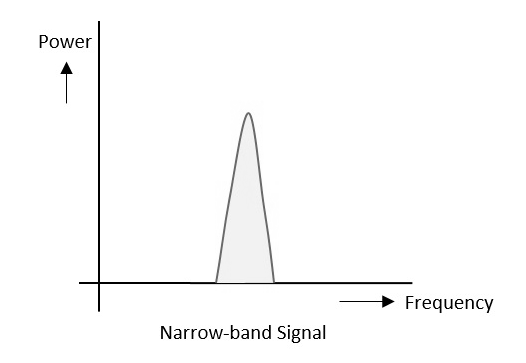Here are the features of narrow-band signals −

• Band of signals occupy narrow range of frequencies.
• Power density is high.
• Spread of energy is low and concentrated.

Though the features are good, these signals are prone to interference.

The spread spectrum signals have the signal strength distributed as shown in the following frequency spectrum figure.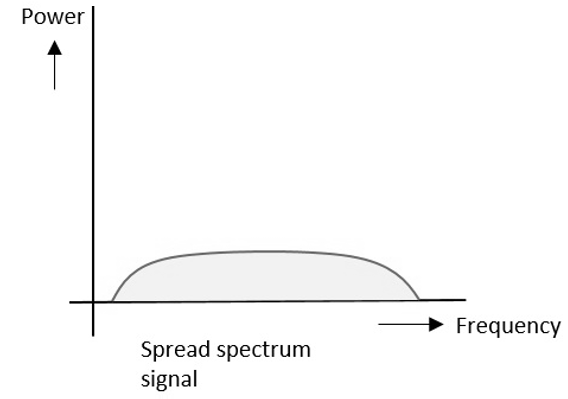Here are the features of spread spectrum signals −

• Band of signals occupy a wide range of frequencies.
• Power density is very low.

With these features, the spread spectrum signals are highly resistant to interference or jamming. Since, multiple users can share the same spread spectrum bandwidth without interfering with one another, these can be called as multiple access techniques.

Spread spectrum multiple access techniques use signals which have a transmission bandwidth whose magnitude is greater than the minimum required RF bandwidth.

Spread spectrum signals can be classified into two categories −

• Frequency Hopped Spread spectrum (FHSS)
• Direct Sequence Spread spectrum (DSSS)

This is frequency hopping technique, where the users are made to change the frequencies of usage, from one to another in a specified time interval, hence it is called as frequency hopping.

For example, a frequency was allotted to sender 1 for a particular period of time. Now, after a while, sender 1 hops to the other frequency and sender 2 uses the first frequency, which was previously used by sender1. This is called as frequency reuse.

The frequencies of the data are hopped from one to another in order to provide secure transmission. The amount of time spent on each frequency hop is called as Dwell time.

Whenever a user wants to send data using this DSSS technique, each and every bit of the user data is multiplied by a secret code, called as chipping code. This chipping code is nothing but the spreading code which is multiplied with the original message and transmitted. The receiver uses the same code to retrieve the original message.

This DSSS is also called as Code Division Multiple Access (CDMA).

## Comparison between FHSS and DSSS/CDMA

Both the spread spectrum techniques are popular for their characteristics. To have a clear understanding, let us take a look at their comparisons.

FHSS DSSS/CDMA
Multiple frequencies are used Single frequency is used
Hard to find the user’s frequency at any instant of time User frequency, once allotted is always the same
Frequency reuse is allowed Frequency reuse is not allowed
The sender need not wait The sender has to wait if the spectrum is busy
Power strength of the signal is high Power strength of the signal is low
It is stronger and penetrates through the obstacles It is weaker compared to FHSS
It is never affected by interference It can be affected by interference
It is cheaper It is expensive
This is the mostly used technique This technique is not frequently used

• Cross-talk elimination
• Better output with data integrity
• Reduced effect of multipath fading
• Better security
• Reduction in noise
• Co-existence with other systems
• Longer operative distances
• Hard to detect
• Hard to demodulate/decode
• Harder to jam the signals

Although spread spectrum techniques were originally designed for military uses, they are now being used widely as commercial purpose.

# Principles of Optical Fiber Communications

The digital communication techniques discussed so far have led to the advancement in the study of both Optical and Satellite communications. Let us take a look at them.

## Fiber Optics

An optical fiber can be understood as a dielectric waveguide, which operates at optical frequencies. The device or a tube, if bent or if terminated to radiate energy, is called a waveguide, in general. Following image depicts a bunch of fiber optic cables.The electromagnetic energy travels through it in the form of light. The light propagation, along a waveguide can be described in terms of a set of guided electromagnetic waves, called as modes of the waveguide.

## Working Principle

A fundamental optical parameter one should have an idea about, while studying fiber optics is Refractive index. By definition, “The ratio of the speed of light in a vacuum to that in matter is the index of refraction n of the material.” It is represented as −

$$n = \frac{c}{v}$$

Where,

c = the speed of light in free space = 3 × 108 m/s

v = the speed of light in di-electric or non-conducting material

Generally, for a travelling light ray, reflection takes place when n2 < n1 . The bent of light ray at the interface is the result of difference in the speed of light in two materials that have different refractive indices. The relationship between these angles at the interface can be termed as Snell’s law. It is represented as −

$$n_1sin\phi _1 = n_2sin\phi _2$$

Where,

$\phi _1$ is the angle of incidence

$\phi _2$ is the refracted angle

n1 and n2 are the refractive indices of two materials

For an optically dense material, if the reflection takes place within the same material, then such a phenomenon is called as internal reflection. The incident angle and refracted angle are shown in the following figure.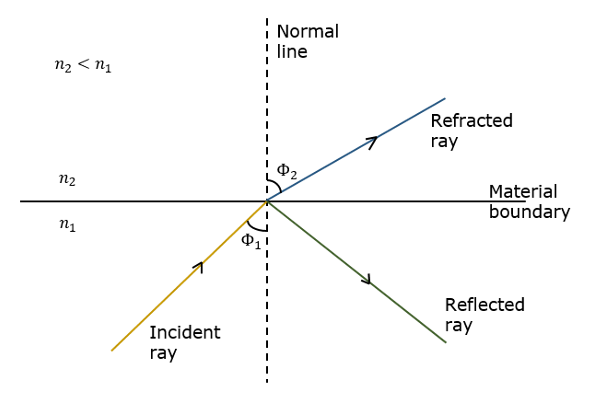If the angle of incidence $\phi _1$ is much larger, then the refracted angle $\phi _2$ at a point becomes Π/2 . Further refraction is not possible beyond this point. Hence, such a point is called as Critical angle $\phi _c$. When the incident angle $\phi _1$ is greater than the critical angle, the condition for total internal reflection is satisfied.

The following figure shows these terms clearly.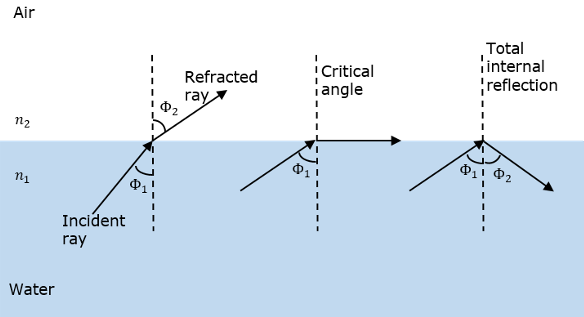A light ray, if passed into a glass, at such condition, it is totally reflected back into the glass with no light escaping from the surface of the glass.

## Parts of a Fiber

The most commonly used optical fiber is single solid di-electric cylinder of radius a and index of refraction n1. The following figure explains the parts of an optical fiber.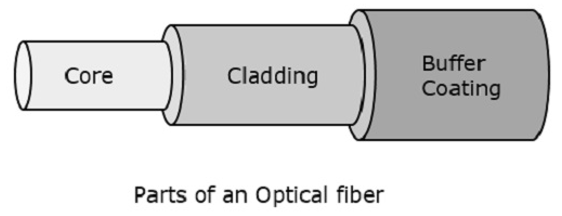This cylinder is known as the Core of the fiber. A solid di-electric material surrounds the core, which is called as Cladding. Cladding has a refractive index n2 which is less than n1.

• Reducing scattering losses.
• Adds mechanical strength to the fiber.
• Protects the core from absorbing unwanted surface contaminants.

## Types of Optical Fibers

Depending upon the material composition of the core, there are two types of fibers used commonly. They are −

• Step-index fiber − The refractive index of the core is uniform throughout and undergoes an abrupt change (or step) at the cladding boundary.

• Graded-index fiber − The core refractive index is made to vary as a function of the radial distance from the center of the fiber.

Both of these are further divided into −

• Single-mode fiber − These are excited with laser.

• Multi-mode fiber − These are excited with LED.

## Optical Fiber Communications

The communication system of fiber optics is well understood by studying the parts and sections of it. The major elements of an optical fiber communication system are shown in the following figure.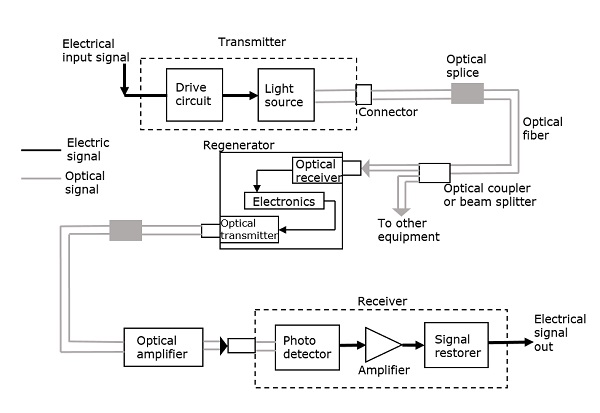The basic components are light signal transmitter, the optical fiber, and the photo detecting receiver. The additional elements such as fiber and cable splicers and connectors, regenerators, beam splitters, and optical amplifiers are employed to improve the performance of the communication system.

The functional advantages of optical fibers are −

• The transmission bandwidth of the fiber optic cables is higher than the metal cables.

• The amount of data transmission is higher in fiber optic cables.

• The power loss is very low and hence helpful in long-distance transmissions.

• Fiber optic cables provide high security and cannot be tapped.

• Fiber optic cables are the most secure way for data transmission.

• Fiber optic cables are immune to electromagnetic interference.

• These are not affected by electrical noise.

The physical advantages of fiber optic cables are −

• The capacity of these cables is much higher than copper wire cables.

• Though the capacity is higher, the size of the cable doesn’t increase like it does in copper wire cabling system.

• The space occupied by these cables is much less.

• The weight of these FOC cables is much lighter than the copper ones.

• Since these cables are di-electric, no spark hazards are present.

• These cables are more corrosion resistant than copper cables, as they are bent easily and are flexible.

• The raw material for the manufacture of fiber optic cables is glass, which is cheaper than copper.

• Fiber optic cables last longer than copper cables.

Although fiber optics offer many advantages, they have the following drawbacks −

• Though fiber optic cables last longer, the installation cost is high.

• The number of repeaters are to be increased with distance.

• They are fragile if not enclosed in a plastic sheath. Hence, more protection is needed than copper ones.

### Applications of Fiber Optics

The optical fibers have many applications. Some of them are as follows −

• Used in telephone systems

• Used in sub-marine cable networks

• Used in data link for computer networks, CATV Systems

• Used in CCTV surveillance cameras

• Used for connecting fire, police, and other emergency services.

• Used in hospitals, schools, and traffic management systems.

• They have many industrial uses and also used for in heavy duty constructions.

# Principles of Satellite Communications

A satellite is a body that moves around another body in a mathematically predictable path called an Orbit. A communication satellite is nothing but a microwave repeater station in space that is helpful in telecommunications, radio, and television along with internet applications.

A repeater is a circuit which increases the strength of the signal it receives and retransmits it. But here this repeater works as a transponder, which changes the frequency band of the transmitted signal, from the received one.

The frequency with which the signal is sent into the space is called Uplink frequency, while the frequency with which it is sent by the transponder is Downlink frequency.

The following figure illustrates this concept clearly.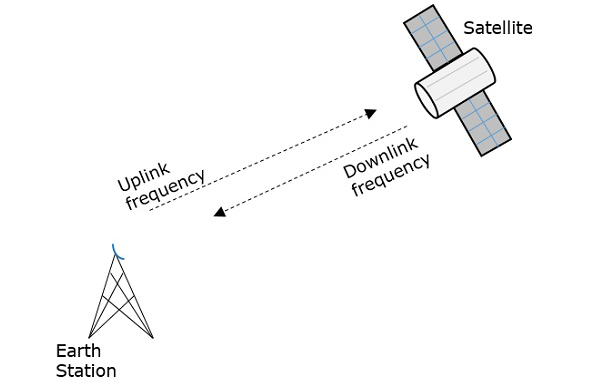Now, let us have a look at the advantages, disadvantages and applications of satellite communications.

There are many Advantages of satellite communications such as −

• Flexibility

• Ease in installing new circuits

• Distances are easily covered and cost doesn’t matter

• Each and every corner of earth is covered

• User can control the network

Satellite communication has the following drawbacks −

• The initial costs such as segment and launch costs are too high.

• Congestion of frequencies

• Interference and propagation

### Satellite Communication − Applications

Satellite communication finds its applications in the following areas −

• In TV broadcasting such as DTH.

• In Internet applications such as providing Internet connection for data transfer, GPS applications, Internet surfing, etc.

• For voice communications.

• For research and development sector, in many areas.

• In military applications and navigations.

The orientation of the satellite in its orbit depends upon the three laws called as Kepler’s laws.

## Kepler’s Laws

Johannes Kepler (1571-1630) the astronomical scientist, gave 3 revolutionary laws, regarding the motion of satellites. The path followed by a satellite around its primary (the earth) is an ellipse. Ellipse has two foci - F1 and F2, the earth being one of them.

If the distance from the center of the object to a point on its elliptical path is considered, then the farthest point of an ellipse from the center is called as apogee and the shortest point of an ellipse from the center is called as perigee.

### Kepler’s 1st Law

Kepler’s 1st law states that, “every planet revolves around the sun in an elliptical orbit, with sun as one of its foci.” As such, a satellite moves in an elliptical path with earth as one of its foci.

The semi major axis of the ellipse is denoted as ‘a’ and semi minor axis is denoted as b. Therefore, the eccentricity e of this system can be written as −

$$e = \frac{\sqrt{a^{2}-b^{2}}}{a}$$

• Eccentricity (e) − It is the parameter which defines the difference in the shape of the ellipse rather than that of a circle.

• Semi-major axis (a) − It is the longest diameter drawn joining the two foci along the center, which touches both the apogees (farthest points of an ellipse from the center).

• Semi-minor axis (b) − It is the shortest diameter drawn through the center which touches both the perigees (shortest points of an ellipse from the center).

These are well described in the following figure.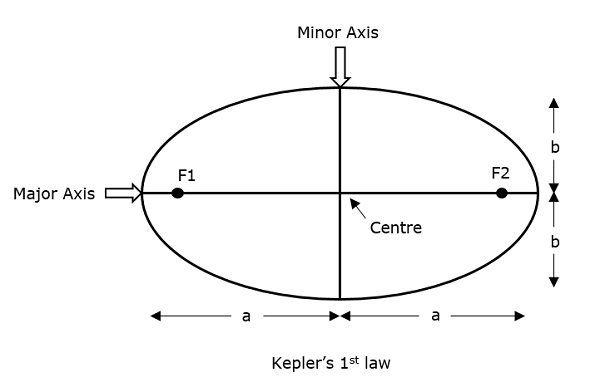For an elliptical path, it is always desirable that the eccentricity should lie in between 0 and 1, i.e. 0 < e < 1 because if e becomes zero, the path will be no more in elliptical shape rather it will be converted into a circular path.

### Kepler’s 2nd Law

Kepler’s 2nd law states that, “For equal intervals of time, the area covered by the satellite is equal with respect to the center of the earth.”

It can be understood by taking a look at the following figure.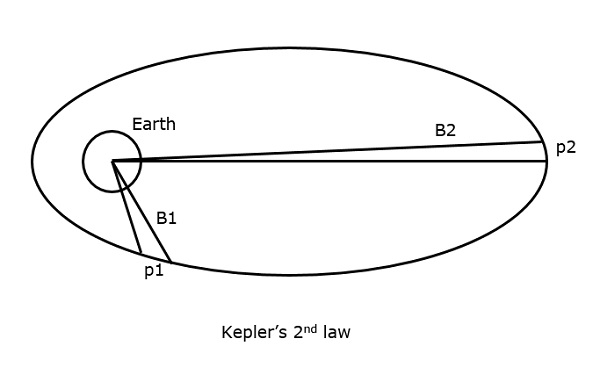Suppose that the satellite covers p1 and p2 distances, in the same time interval, then the areas B1 and B2 covered in both instances respectively, are equal.

### Kepler’s 3rd Law

Kepler’s 3rd law states that, “The square of the periodic time of the orbit is proportional to the cube of the mean distance between the two bodies.”

This can be written mathematically as

$$T^{2}\:\alpha\:\:a^{3}$$

Which implies

$$T^{2} = \frac{4\pi ^{2}}{GM}a^{3}$$

Where $\frac{4\pi ^{2}}{GM}$ is the proportionality constant (according to Newtonian Mechanics)

$$T^{2} = \frac{4\pi ^{2}}{\mu}a^{3}$$

Where μ = the earth’s geocentric gravitational constant, i.e. Μ = 3.986005 × 1014 m3/sec2

$$1 = \left ( \frac{2\pi}{T} \right )^{2}\frac{a^{3}}{\mu}$$

$$1 = n^{2}\frac{a^{3}}{\mu}\:\:\:\Rightarrow \:\:\:a^{3} = \frac{\mu}{n^{2}}$$

Where n = the mean motion of the satellite in radians per second

The orbital functioning of satellites is calculated with the help of these Kepler’s laws.

Along with these, there is an important thing which has to be noted. A satellite, when it revolves around the earth, undergoes a pulling force from the earth which is the gravitational force. Also, it experiences some pulling force from the sun and the moon. Hence, there are two forces acting on it. They are −

• Centripetal force − The force that tends to draw an object moving in a trajectory path, towards itself is called as centripetal force.

• Centrifugal force − The force that tends to push an object moving in a trajectory path, away from its position is called as centrifugal force.

So, a satellite has to balance these two forces to keep itself in its orbit.

## Earth Orbits

A satellite when launched into space, needs to be placed in a certain orbit to provide a particular way for its revolution, so as to maintain accessibility and serve its purpose whether scientific, military, or commercial. Such orbits which are assigned to satellites, with respect to earth are called as Earth Orbits. The satellites in these orbits are Earth Orbit Satellites.

The important kinds of Earth Orbits are −

• Geo Synchronous Earth Orbit

• Medium Earth Orbit

• Low Earth Orbit

## Geosynchronous Earth Orbit Satellites

A Geo-Synchronous Earth Orbit (GEO) satellite is one which is placed at an altitude of 22,300 miles above the Earth. This orbit is synchronized with a side real day (i.e., 23hours 56minutes). This orbit can have inclination and eccentricity. It may not be circular. This orbit can be tilted at the poles of the earth. But it appears stationary when observed from the Earth.

The same geo-synchronous orbit, if it is circular and in the plane of equator, it is called as geo-stationary orbit. These satellites are placed at 35,900kms (same as geosynchronous) above the Earth’s Equator and they keep on rotating with respect to earth’s direction (west to east). These satellites are considered stationary with respect to earth and hence the name implies.

Geo-Stationary Earth Orbit Satellites are used for weather forecasting, satellite TV, satellite radio and other types of global communications.

The following figure shows the difference between Geo-synchronous and Geo-stationary orbits. The axis of rotation indicates the movement of Earth.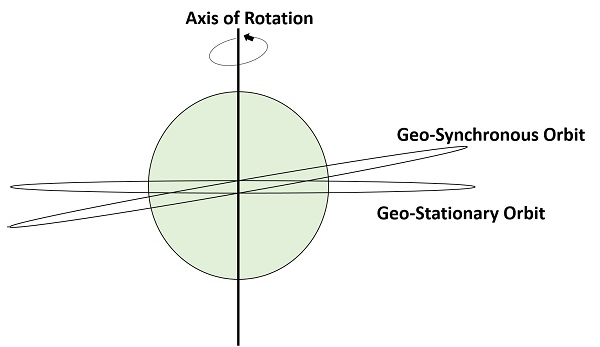Note − Every geo-stationary orbit is a geo-synchronous orbit. But every geo-synchronous orbit is NOT a Geo-stationary orbit.

## Medium Earth Orbit Satellites

Medium Earth Orbit (MEO) satellite networks will orbit at distances of about 8000 miles from the earth's surface. Signals transmitted from a MEO satellite travel a shorter distance. This translates to improved signal strength at the receiving end. This shows that smaller, more lightweight receiving terminals can be used at the receiving end.

Since the signal is travelling a shorter distance to and from the satellite, there is less transmission delay. Transmission delay can be defined as the time it takes for a signal to travel up to a satellite and back down to a receiving station.

For real-time communications, the shorter the transmission delay, the better will be the communication system. As an example, if a GEO satellite requires 0.25 seconds for a round trip, then MEO satellite requires less than 0.1 seconds to complete the same trip. MEOs operates in the frequency range of 2 GHz and above.

## Low Earth Orbit Satellites

The Low Earth Orbit (LEO) satellites are mainly classified into three categories namely, little LEOs, big LEOs, and Mega-LEOs. LEOs will orbit at a distance of 500 to 1000 miles above the earth's surface.

This relatively short distance reduces transmission delay to only 0.05 seconds. This further reduces the need for sensitive and bulky receiving equipment. Little LEOs will operate in the 800 MHz (0.8 GHz) range. Big LEOs will operate in the 2 GHz or above range, and Mega-LEOs operates in the 20-30 GHz range.

The higher frequencies associated with Mega-LEOs translates into more information carrying capacity and yields to the capability of real-time, low delay video transmission scheme.

The following figure depicts the paths of LEO, MEO, and GEO.